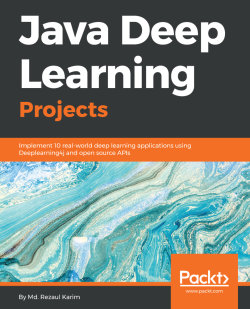### Java Deep Learning Projects5 (1 reviews total)
By Md. Rezaul Karim
• Start FREE trial Subscribe Access now
• \$39.99 eBook

• Constantly updated with 100+ new titles each month
• Breadth and depth in over 1,000+ technologies

Java is one of the most widely used programming languages. With the rise of deep learning, it has become a popular choice of tool among data scientists and machine learning experts.

Java Deep Learning Projects starts with an overview of deep learning concepts and then delves into advanced projects. You will see how to build several projects using different deep neural network architectures such as multilayer perceptrons, Deep Belief Networks, CNN, LSTM, and Factorization Machines.

You will get acquainted with popular deep and machine learning libraries for Java such as Deeplearning4j, Spark ML, and RankSys and you’ll be able to use their features to build and deploy projects on distributed computing environments.

You will then explore advanced domains such as transfer learning and deep reinforcement learning using the Java ecosystem, covering various real-world domains such as healthcare, NLP, image classification, and multimedia analytics with an easy-to-follow approach. Expert reviews and tips will follow every project to give you insights and hacks.

By the end of this book, you will have stepped up your expertise when it comes to deep learning in Java, taking it beyond theory and be able to build your own advanced deep learning systems.

Publication date:
June 2018
Publisher
Packt
Pages
436
ISBN
9781788997454

## Chapter 1. Getting Started with Deep Learning

In this chapter, we will explain some basic concepts of Machine Learning (ML) and Deep Learning (DL) that will be used in all subsequent chapters. We will start with a brief introduction to ML. Then we will move on to DL, which is one of the emerging branches of ML.

We will briefly discuss some of the most well-known and widely used neural network architectures. Next, we will look at various features of deep learning frameworks and libraries. Then we will see how to prepare a programming environment, before moving on to coding with some open source, deep learning libraries such as DeepLearning4J (DL4J)

Then we will solve a very famous ML problem: the Titanic survival prediction. For this, we will use an Apache Spark-based Multilayer Perceptron (MLP) classifier to solve this problem. Finally, we'll see some frequently asked questions that will help us generalize our basic understanding of DL. Briefly, the following topics will be covered:

• A soft introduction to ML
• Artificial Neural Networks (ANNs)
• Deep neural network architectures
• Deep learning frameworks
• Deep learning from disasters—Titanic survival prediction using MLP

## A soft introduction to ML

ML approaches are based on a set of statistical and mathematical algorithms in order to carry out tasks such as classification, regression analysis, concept learning, predictive modeling, clustering, and mining of useful patterns. Thus, with the use of ML, we aim at improving the learning experience such that it becomes automatic. Consequently, we may not need complete human interactions, or at least we can reduce the level of such interactions as much as possible.

### Working principles of ML algorithms

We now refer to a famous definition of ML by Tom M. Mitchell (Machine Learning, Tom Mitchell, McGraw Hill), where he explained what learning really means from a computer science perspective:

"A computer program is said to learn from experience E with respect to some class of tasks T and performance measure P, if its performance at tasks in T, as measured by P, improves with experience E."

Based on this definition, we can conclude that a computer program or machine can do the following:

• Learn from data and histories
• Improve with experience
• Iteratively enhance a model that can be used to predict outcomes of questions

Since they are at the core of predictive analytics, almost every ML algorithm we use can be treated as an optimization problem. This is about finding parameters that minimize an objective function, for example, a weighted sum of two terms like a cost function and regularization. Typically, an objective function has two components:

• A regularizer, which controls the complexity of the model
• The loss, which measures the error of the model on the training data.

On the other hand, the regularization parameter defines the trade-off between minimizing the training error and the model's complexity in an effort to avoid overfitting problems. Now, if both of these components are convex, then their sum is also convex; it is non-convex otherwise. More elaborately, when using an ML algorithm, the goal is to obtain the best hyperparameters of a function that return the minimum error when making predictions. Therefore, using a convex optimization technique, we can minimize the function until it converges towards the minimum error.

Given that a problem is convex, it is usually easier to analyze the asymptotic behavior of the algorithm, which shows how fast it converges as the model observes more and more training data. The challenge of ML is to allow training a model so that it can recognize complex patterns and make decisions not only in an automated way but also as intelligently as possible. The entire learning process requires input datasets that can be split (or are already provided) into three types, outlined as follows:

• A training set is the knowledge base coming from historical or live data used to fit the parameters of the ML algorithm. During the training phase, the ML model utilizes the training set to find optimal weights of the network and reach the objective function by minimizing the training error. Here, the back-prop rule (or another more advanced optimizer with a proper updater; we'll see this later on) is used to train the model, but all the hyperparameters are need to be set before the learning process starts.
• A validation set is a set of examples used to tune the parameters of an ML model. It ensures that the model is trained well and generalizes towards avoiding overfitting. Some ML practitioners refer to it as a development set or dev set as well.
• A test set is used for evaluating the performance of the trained model on unseen data. This step is also referred to as model inferencing. After assessing the final model on the test set (that is, when we're fully satisfied with the model's performance), we do not have to tune the model any further but the trained model can be deployed in a production-ready environment.

A common practice is splitting the input data (after necessary pre-processing and feature engineering) into 60% for training, 10% for validation, and 20% for testing, but it really depends on use cases. Also, sometimes we need to perform up-sampling or down-sampling on the data based on the availability and quality of the datasets.

Moreover, the learning theory uses mathematical tools that derive from probability theory and information theory. Three learning paradigms will be briefly discussed:

• Supervised learning
• Unsupervised learning
• Reinforcement learning

The following diagram summarizes the three types of learning, along with the problems they address: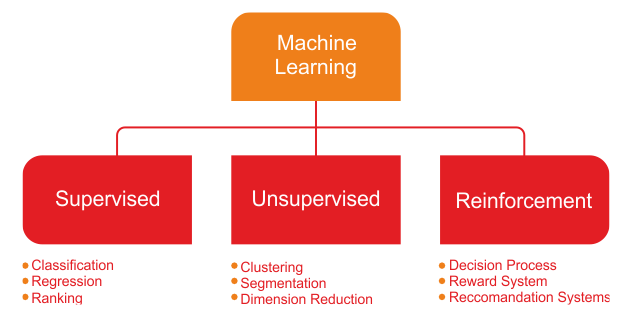Types of learning and related problems

### Supervised learning

Supervised learning is the simplest and most well-known automatic learning task. It is based on a number of pre-defined examples, in which the category to which each of the inputs should belong is already known. Figure 2 shows a typical workflow of supervised learning.

An actor (for example, an ML practitioner, data scientist, data engineer, ML engineer, and so on) performs Extraction Transformation Load (ETL) and the necessary feature engineering (including feature extraction, selection, and so on) to get the appropriate data having features and labels. Then he does the following:

1. Splits the data into training, development, and test sets
2. Uses the training set to train an ML model
1. The validation set is used to validate the training against the overfitting problem and regularization
2. He then evaluates the model's performance on the test set (that is unseen data)
4. Finally, he deploys the best model in a production-ready environment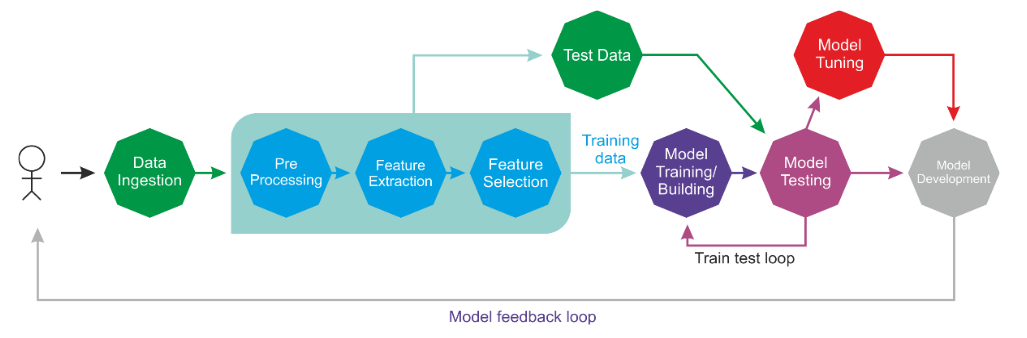Supervised learning in action

In the overall life cycle, there might be many actors involved (for example, a data engineer, data scientist, or ML engineer) to perform each step independently or collaboratively.

The supervised learning context includes classification and regression tasks; classification is used to predict which class a data point is part of (discrete value), while regression is used to predict continuous values. In other words, a classification task is used to predict the label of the class attribute, while a regression task is used to make a numeric prediction of the class attribute.

In the context of supervised learning, unbalanced data refers to classification problems where we have unequal instances for different classes. For example, if we have a classification task for only two classes, balanced data would mean 50% pre-classified examples for each of the classes.

If the input dataset is a little unbalanced (for example, 60% data points for one class and 40% for the other class), the learning process will require for the input dataset to be split randomly into three sets, with 50% for the training set, 20% for the validation set, and the remaining 30% for the testing set.

### Unsupervised learning

In unsupervised learning, an input set is supplied to the system during the training phase. In contrast with supervised learning, the input objects are not labeled with their class. For classification, we assumed that we are given a training dataset of correctly labeled data. Unfortunately, we do not always have that advantage when we collect data in the real world.

For example, let's say you have a large collection of totally legal, not pirated, MP3 files in a crowded and massive folder on your hard drive. In such a case, how could we possibly group songs together if we do not have direct access to their metadata? One possible approach could be to mix various ML techniques, but clustering is often the best solution.

Now, what if you can build a clustering predictive model that helps automatically group together similar songs and organize them into your favorite categories, such as country, rap, rock, and so on? In short, unsupervised learning algorithms are commonly used in clustering problems. The following diagram gives us an idea of a clustering technique applied to solve this kind of problem: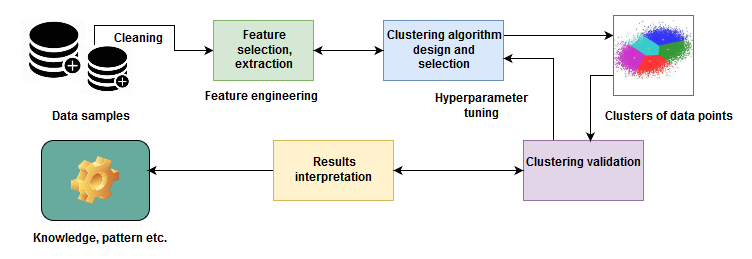Clustering techniques – an example of unsupervised learning

Although the data points are not labeled, we can still do the necessary feature engineering and grouping of a set of objects in such a way that objects in the same group (called a cluster) are brought together. This is not easy for a human. Rather, a standard approach is to define a similarity measure between two objects and then look for any cluster of objects that are more similar to each other than they are to the objects in the other clusters. Once we've done the clustering of the data points (that is, MP3 files) and the validation is completed, we know the pattern of the data (that is, what type of MP3 files fall in which group).

### Reinforcement learning

Reinforcement learning is an artificial intelligence approach that focuses on the learning of the system through its interactions with the environment. In reinforcement learning, the system's parameters are adapted based on the feedback obtained from the environment, which in turn provides feedback on the decisions made by the system. The following diagram shows a person making decisions in order to arrive at their destination.

Let's take an example of the route you take from home to work. In this case, you take the same route to work every day. However, out of the blue, one day you get curious and decide to try a different route with a view to finding the shortest path. This dilemma of trying out new routes or sticking to the best-known route is an example of exploration versus exploitation: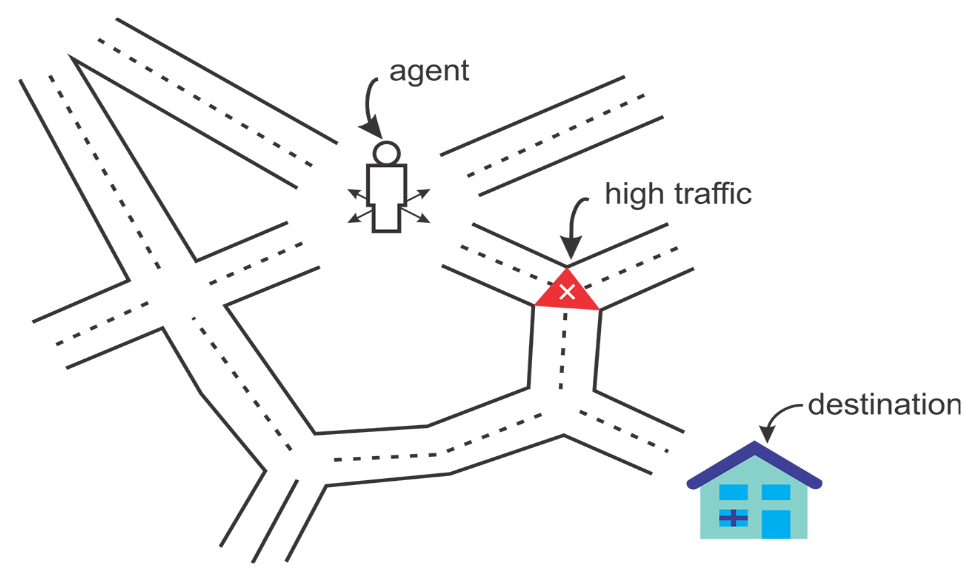An agent always tries to reach the destination

We can take a look at one more example in terms of a system modeling a chess player. In order to improve its performance, the system utilizes the result of its previous moves; such a system is said to be a system learning with reinforcement.

We have seen the basic working principles of ML algorithms. Then we have seen what the basic ML tasks are and how they formulate domain-specific problems. Now let's take a look at how can we summarize ML tasks and some applications in the following diagram: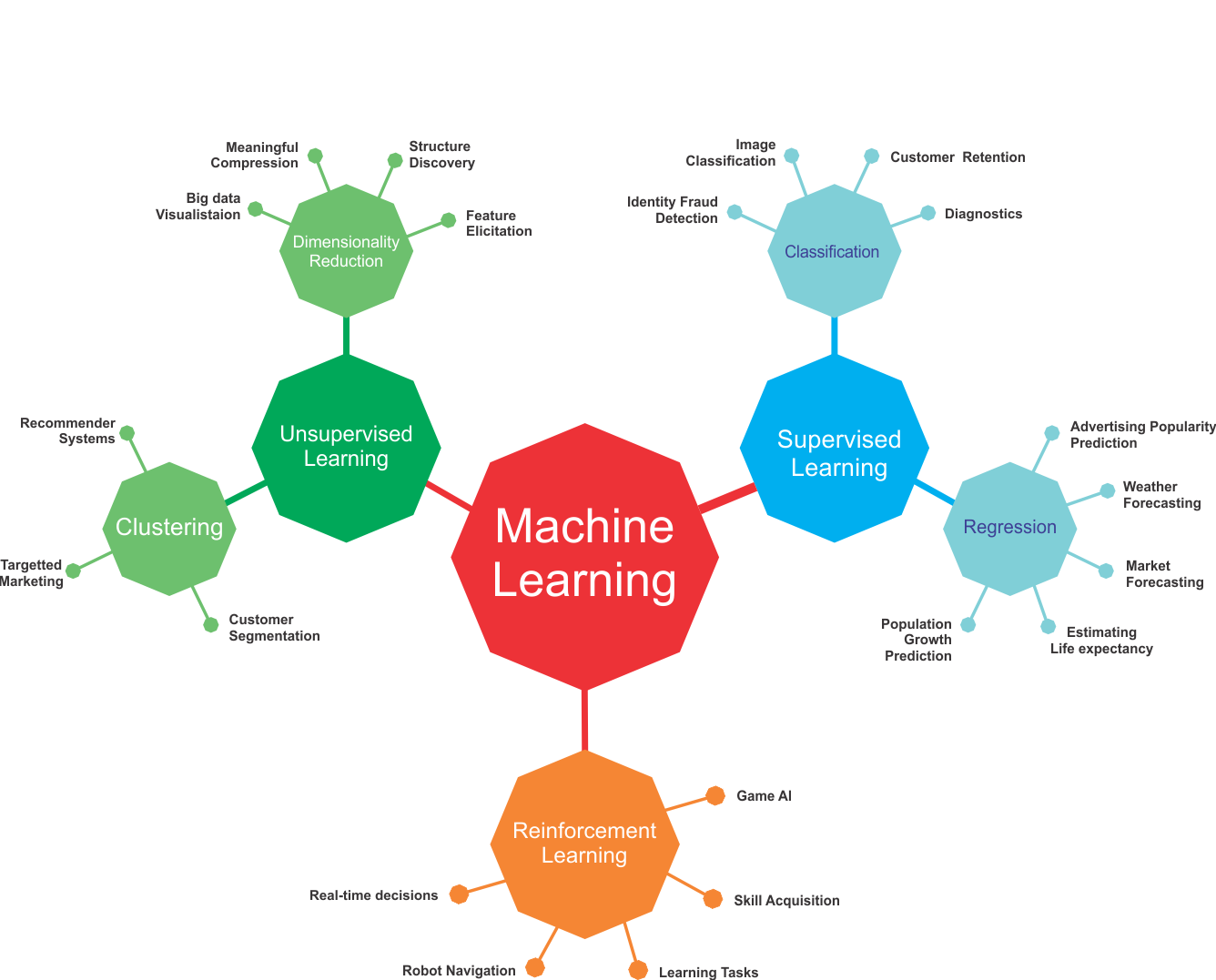ML tasks and some use cases from different application domains

However, the preceding figure lists only a few use cases and applications using different ML tasks. In practice, ML is used in numerous use cases and applications. We will try to cover a few of those throughout this book.

## Delving into deep learning

Simple ML methods that were used in normal-size data analysis are not effective anymore and should be substituted by more robust ML methods. Although classical ML techniques allow researchers to identify groups or clusters of related variables, the accuracy and effectiveness of these methods diminish with large and high-dimensional datasets.

Here comes deep learning, which is one of the most important developments in artificial intelligence in the last few years. Deep learning is a branch of ML based on a set of algorithms that attempt to model high-level abstractions in data.

### How did DL take ML into next level?

In short, deep learning algorithms are mostly a set of ANNs that can make better representations of large-scale datasets, in order to build models that learn these representations very extensively. Nowadays it's not limited to ANNs, but there have been really many theoretical advances and software and hardware improvements that were necessary for us to get to this day. In this regard, Ian Goodfellow et al. (Deep Learning, MIT Press, 2016) defined deep learning as follows:

"Deep learning is a particular kind of machine learning that achieves great power and flexibility by learning to represent the world as a nested hierarchy of concepts, with each concept defined in relation to simpler concepts, and more abstract representations computed in terms of less abstract ones."

Let's take an example; suppose we want to develop a predictive analytics model, such as an animal recognizer, where our system has to resolve two problems:

• To classify whether an image represents a cat or a dog
• To cluster images of dogs and cats.

If we solve the first problem using a typical ML method, we must define the facial features (ears, eyes, whiskers, and so on) and write a method to identify which features (typically nonlinear) are more important when classifying a particular animal.

However, at the same time, we cannot address the second problem because classical ML algorithms for clustering images (such as k-means) cannot handle nonlinear features. Deep learning algorithms will take these two problems one step further and the most important features will be extracted automatically after determining which features are the most important for classification or clustering.

In contrast, when using a classical ML algorithm, we would have to provide the features manually. In summary, the deep learning workflow would be as follows:

• A deep learning algorithm would first identify the edges that are most relevant when clustering cats or dogs. It would then try to find various combinations of shapes and edges hierarchically. This step is called ETL.
• After several iterations, hierarchical identification of complex concepts and features is carried out. Then, based on the identified features, the DL algorithm automatically decides which of these features are most significant (statistically) to classify the animal. This step is feature extraction.
• Finally, it takes out the label column and performs unsupervised training using AutoEncoders (AEs) to extract the latent features to be redistributed to k-means for clustering.
• Then the clustering assignment hardening loss (CAH loss) and reconstruction loss are jointly optimized towards optimal clustering assignment. Deep Embedding Clustering (see more at https://arxiv.org/pdf/1511.06335.pdf) is an example of such an approach. We will discuss deep learning-based clustering approaches in Chapter 11, Discussion, Current Trends, and Outlook

Up to this point, we have seen that deep learning systems are able to recognize what an image represents. A computer does not see an image as we see it because it only knows the position of each pixel and its color. Using deep learning techniques, the image is divided into various layers of analysis.

At a lower level, the software analyzes, for example, a grid of a few pixels with the task of detecting a type of color or various nuances. If it finds something, it informs the next level, which at this point checks whether or not that given color belongs to a larger form, such as a line. The process continues to the upper levels until you understand what is shown in the image. The following diagram shows what we have discussed in the case of an image classification system: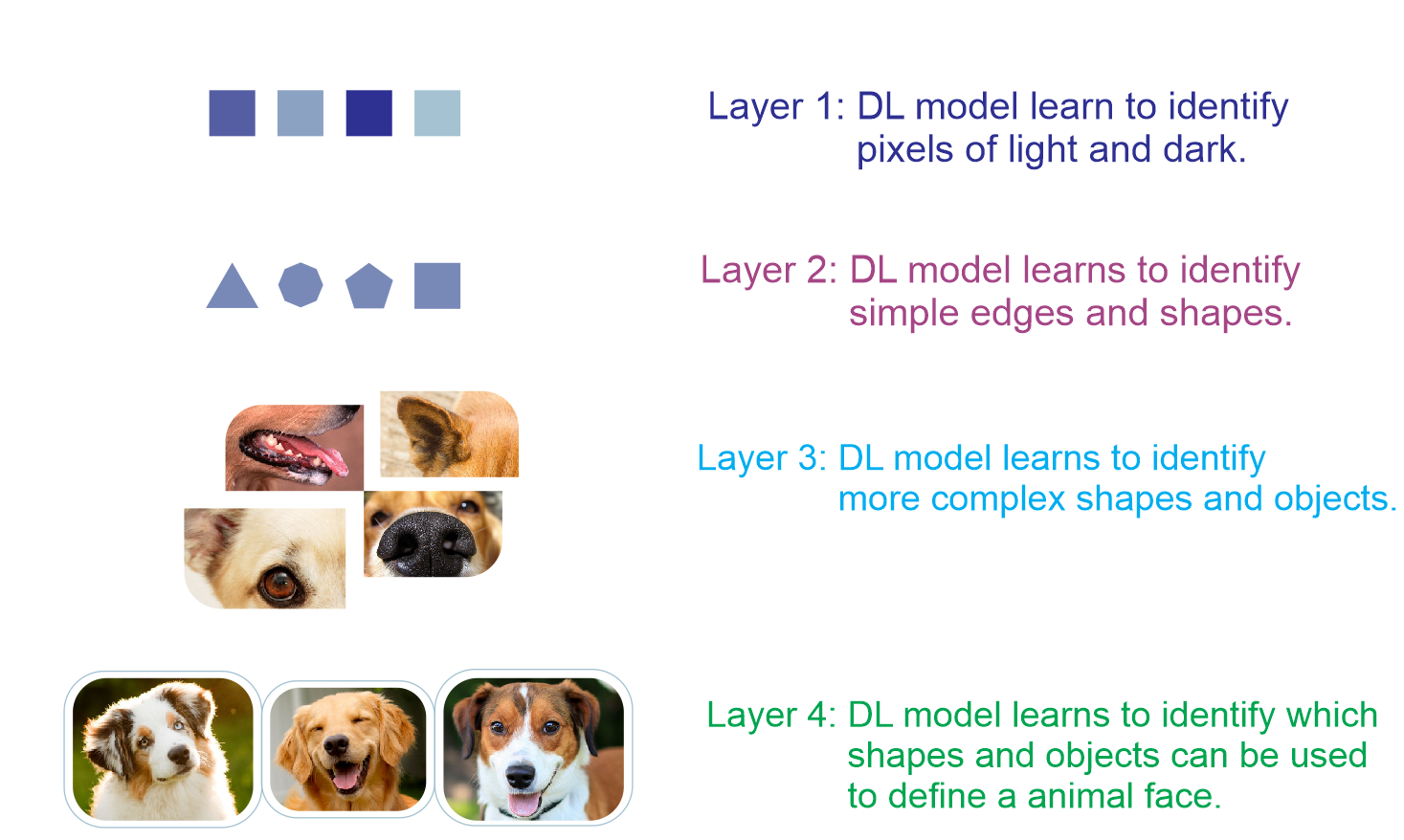A deep learning system at work on a dog versus cat classification problem

More precisely, the preceding image classifier can be built layer by layer, as follows:

• Layer 1: The algorithm starts identifying the dark and light pixels from the raw images
• Layer 2: The algorithm then identifies edges and shapes
• Layer 3: It then learns more complex shapes and objects
• Layer 4: The algorithm then learns which objects define a human face

Although this is a very simple classifier, software capable of doing these types of things is now widespread and is found in systems for recognizing faces, or in those for searching by an image on Google, for example. These pieces of software are based on deep learning algorithms.

On the contrary, by using a linear ML algorithm, we cannot build such applications since these algorithms are incapable of handling nonlinear image features. Also, using ML approaches, we typically handle a few hyperparameters only. However, when neural networks are brought to the party, things become too complex. In each layer, there are millions or even billions of hyperparameters to tune, so much that the cost function becomes non-convex.

Another reason is that activation functions used in hidden layers are nonlinear, so the cost is non-convex. We will discuss this phenomenon in more detail in later chapters but let's take a quick look at ANNs.

## Artificial Neural Networks

ANNs work on the concept of deep learning. They represent the human nervous system in how the nervous system consists of a number of neurons that communicate with each other using axons.

### Biological neurons

The working principles of ANNs are inspired by how a human brain works, depicted in Figure 7. The receptors receive the stimuli either internally or from the external world; then they pass the information into the biological neurons for further processing. There are a number of dendrites, in addition to another long extension called the axon.

Towards its extremity, there are minuscule structures called synaptic terminals, used to connect one neuron to the dendrites of other neurons. Biological neurons receive short electrical impulses called signals from other neurons, and in response, they trigger their own signals: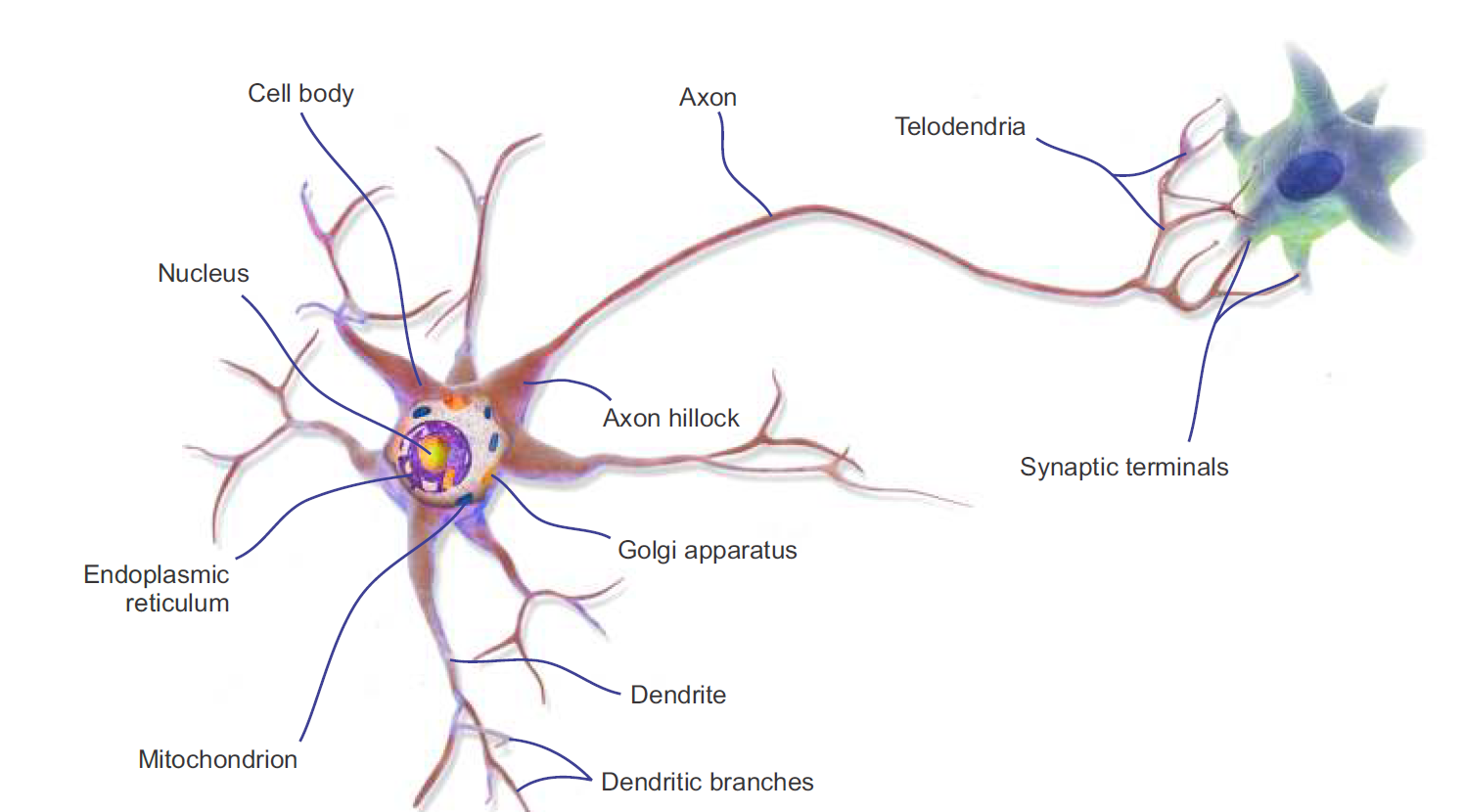Working principle of biological neurons

We can thus summarize that the neuron comprises a cell body (also known as the soma), one or more dendrites for receiving signals from other neurons, and an axon for carrying out the signals generated by the neurons.

A neuron is in an active state when it is sending signals to other neurons. However, when it is receiving signals from other neurons, it is in an inactive state. In an idle state, a neuron accumulates all the signals received before reaching a certain activation threshold. This whole thing motivated researchers to introduce an ANN.

### A brief history of ANNs

Inspired by the working principles of biological neurons, Warren McCulloch and Walter Pitts proposed the first artificial neuron model in 1943 in terms of a computational model of nervous activity. This simple model of a biological neuron, also known as an artificial neuron (AN), has one or more binary (on/off) inputs and one output only.

An AN simply activates its output when more than a certain number of its inputs are active. For example, here we see a few ANNs that perform various logical operations. In this example, we assume that a neuron is activated only when at least two of its inputs are active: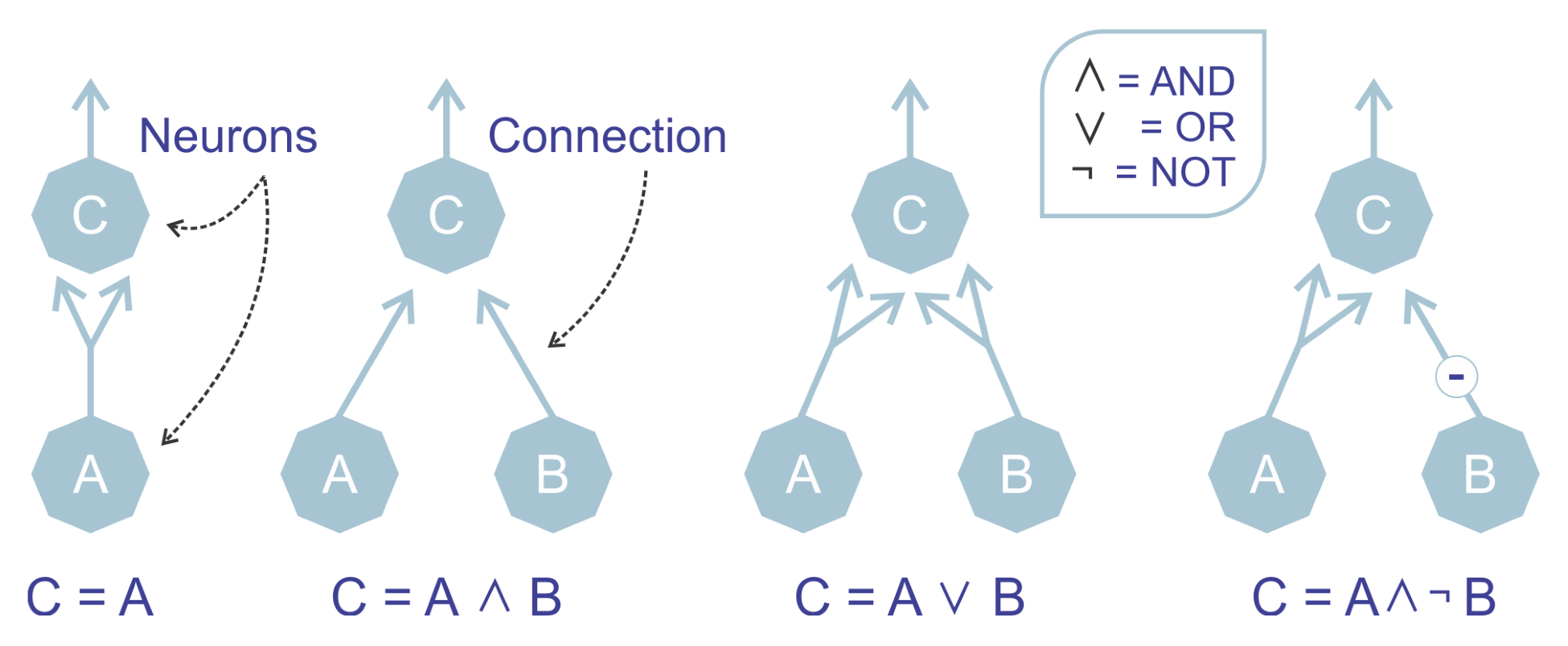ANNs performing simple logical computations

The example sounds too trivial, but even with such a simplified model, it is possible to build a network of ANs. Nevertheless, these networks can be combined to compute complex logical expressions too. This simplified model inspired John von Neumann, Marvin Minsky, Frank Rosenblatt, and many others to come up with another model called a perceptron back in 1957.

The perceptron is one of the simplest ANN architectures we've seen in the last 60 years. It is based on a slightly different AN called a Linear Threshold Unit (LTU). The only difference is that the inputs and outputs are now numbers instead of binary on/off values. Each input connection is associated with a weight. The LTU computes a weighted sum of its inputs, then applies a step function (which resembles the action of an activation function) to that sum, and outputs the result: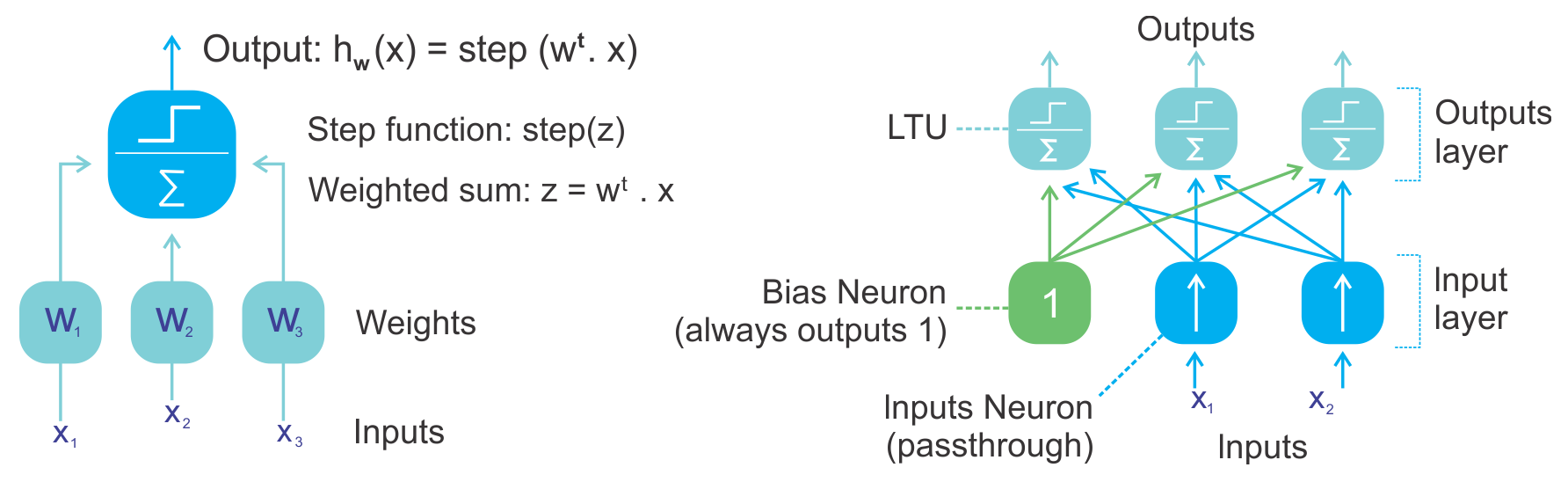The left-side figure represents an LTU and the right-side figure shows a perceptron

One of the downsides of a perceptron is that its decision boundary is linear. Therefore, they are incapable of learning complex patterns. They are also incapable of solving some simple problems like Exclusive OR (XOR). However, later on, the limitations of perceptrons were somewhat eliminated by stacking multiple perceptrons, called MLP.

### How does an ANN learn?

Based on the concept of biological neurons, the term and the idea of ANs arose. Similarly to biological neurons, the artificial neuron consists of the following:

• One or more incoming connections that aggregate signals from neurons
• One or more output connections for carrying the signal to the other neurons
• An activation function, which determines the numerical value of the output signal

The learning process of a neural network is configured as an iterative process of optimization of the weights (see more in the next section). The weights are updated in each epoch. Once the training starts, the aim is to generate predictions by minimizing the loss function. The performance of the network is then evaluated on the test set.

Now we know the simple concept of an artificial neuron. However, generating only some artificial signals is not enough to learn a complex task. Albeit, a commonly used supervised learning algorithm is the backpropagation algorithm, which is very commonly used to train a complex ANN.

## ANNs and the backpropagation algorithm

The backpropagation algorithm aims to minimize the error between the current and the desired output. Since the network is feedforward, the activation flow always proceeds forward from the input units to the output units.

The gradient of the cost function is backpropagated and the network weights get updated; the overall method can be applied to any number of hidden layers recursively. In such a method, the incorporation between two phases is important. In short, the basic steps of the training procedure are as follows:

1. Initialize the network with some random (or more advanced XAVIER) weights
2. For all training cases, follow the steps of forward and backward passes as outlined next

### Forward and backward passes

In the forward pass, a number of operations are performed to obtain some predictions or scores. In such an operation, a graph is created, connecting all dependent operations in a top-to-bottom fashion. Then the network's error is computed, which is the difference between the predicted output and the actual output.

On the other hand, the backward pass is involved mainly with mathematical operations, such as creating derivatives for all differential operations (that is auto-differentiation methods), top to bottom (for example, measuring the loss function to update the network weights), for all the operations in the graph, and then using them in chain rule.

In this pass, for all layers starting with the output layer back to the input layer, it shows the network layer's output with the correct input (error function). Then it adapts the weights in the current layer to minimize the error function. This is backpropagation's optimization step. By the way, there are two types of auto-differentiation methods:

1. Reverse mode: Derivation of a single output with respect to all inputs
2. Forward mode: Derivation of all outputs with respect to one input

The backpropagation algorithm processes the information in such a way that the network decreases the global error during the learning iterations; however, this does not guarantee that the global minimum is reached. The presence of hidden units and the nonlinearity of the output function mean that the behavior of the error is very complex and has many local minimas.

This backpropagation step is typically performed thousands or millions of times, using many training batches, until the model parameters converge to values that minimize the cost function. The training process ends when the error on the validation set begins to increase, because this could mark the beginning of a phase overfitting.

### Weights and biases

Besides the state of a neuron, synaptic weight is considered, which influences the connection within the network. Each weight has a numerical value indicated by Wij, which is the synaptic weight connecting neuron i to neuron j.

### Note

Synaptic weight: This concept evolved from biology and refers to the strength or amplitude of a connection between two nodes, corresponding in biology to the amount of influence the firing of one neuron has on another.

For each neuron (also known as, unit) i, an input vector can be defined by xi= (x1, x2,...xn) and a weight vector can be defined by wi= (wi1, wi2,...win). Now, depending on the position of a neuron, the weights and the output function determine the behavior of an individual neuron. Then during forward propagation, each unit in the hidden layer gets the following signal:Nevertheless, among the weights, there is also a special type of weight called bias unit b. Technically, bias units aren't connected to any previous layer, so they don't have true activity. But still, the bias b value allows the neural network to shift the activation function to the left or right. Now, taking the bias unit into consideration, the modified network output can be formulated as follows:The preceding equation signifies that each hidden unit gets the sum of inputs multiplied by the corresponding weight—summing junction. Then the resultant in the summing junction is passed through the activation function, which squashes the output as depicted in the following figure: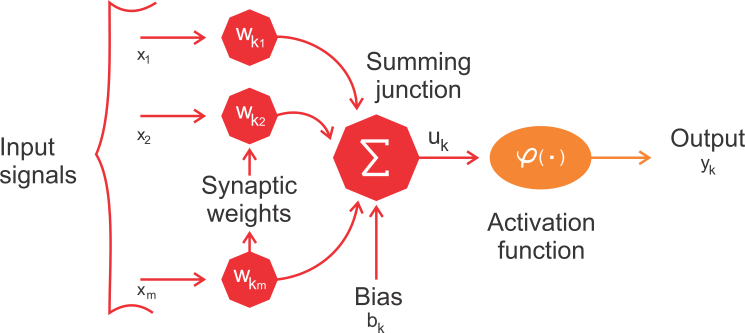Artificial neuron model

Now, a tricky question: how do we initialize the weights? Well, if we initialize all weights to the same value (for example, 0 or 1), each hidden neuron will get exactly the same signal. Let's try to break it down:

• If all weights are initialized to 1, then each unit gets a signal equal to the sum of the inputs
• If all weights are 0, which is even worse, every neuron in a hidden layer will get zero signal

For network weight initialization, Xavier initialization is nowadays used widely. It is similar to random initialization but often turns out to work much better since it can automatically determine the scale of initialization based on the number of input and output neurons.

### Note

Interested readers should refer to this publication for detailed info: Xavier Glorot and Yoshua Bengio, Understanding the difficulty of training deep feedforward neural networks: proceedings of the 13th international conference on Artificial Intelligence and Statistics (AISTATS) 2010, Chia Laguna Resort, Sardinia, Italy; Volume 9 of JMLR: W&CP.

You may be wondering whether you can get rid of random initialization while training a regular DNN (for example, MLP or DBN). Well, recently, some researchers have been talking about random orthogonal matrix initializations that perform better than just any random initialization for training DNNs.

When it comes to initializing the biases, we can initialize them to be zero. But setting the biases to a small constant value such as 0.01 for all biases ensures that all Rectified Linear Unit (ReLU) units can propagate some gradient. However, it neither performs well nor shows consistent improvement. Therefore, sticking with zero is recommended.

#### Weight optimization

Before the training starts, the network parameters are set randomly. Then to optimize the network weights, an iterative algorithm called Gradient Descent (GD) is used. Using GD optimization, our network computes the cost gradient based on the training set. Then, through an iterative process, the gradient G of the error function E is computed.

In following graph, gradient G of error function E provides the direction in which the error function with current values has the steeper slope. Since the ultimate target is to reduce the network error, GD makes small steps in the opposite direction -G. This iterative process is executed a number of times, so the error E would move down towards the global minima. This way, the ultimate target is to reach a point where G = 0, where no further optimization is possible: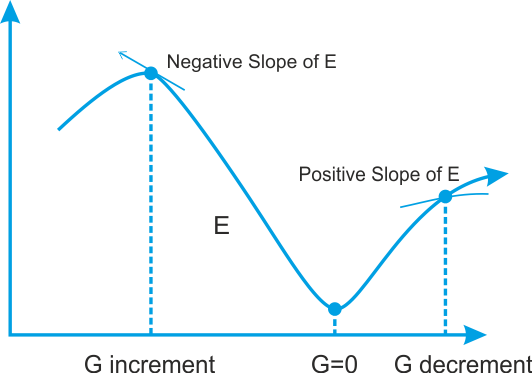Searching for the minimum for the error function E; we move in the direction in which the gradient G of E is minimal

The downside is that it takes too long to converge, which makes it impossible to meet the demand of handling large-scale training data. Therefore, a faster GD called Stochastic Gradient Descent (SDG) is proposed, which is also a widely used optimizer in DNN training. In SGD, we use only one training sample per iteration from the training set to update the network parameters.

### Note

I'm not saying SGD is the only available optimization algorithm, but there are so many advanced optimizers available nowadays, for example, Adam, RMSProp, ADAGrad, Momentum, and so on. More or less, most of them are either direct or indirect optimized versions of SGD.

By the way, the term stochastic comes from the fact that the gradient based on a single training sample per iteration is a stochastic approximation of the true cost gradient.

#### Activation functions

To allow a neural network to learn complex decision boundaries, we apply a non-linear activation function to some of its layers. Commonly used functions include Tanh, ReLU, softmax, and variants of these. More technically, each neuron receives as input signal the weighted sum of the synaptic weights and the activation values of the neurons connected. One of the most widely used functions for this purpose is the so-called sigmoid function. It is a special case of the logistic function, which is defined by the following formula:The domain of this function includes all real numbers, and the co-domain is (0, 1). This means that any value obtained as an output from a neuron (as per the calculation of its activation state), will always be between zero and one. The sigmoid function, as represented in the following diagram, provides an interpretation of the saturation rate of a neuron, from not being active (= 0) to complete saturation, which occurs at a predetermined maximum value (= 1).

On the other hand, a hyperbolic tangent, or tanh, is another form of the activation function. Tanh squashes a real-valued number to the range [-1, 1]. In particular, mathematically, tanh activation function can be expressed as follows:The preceding equation can be represented in the following figure: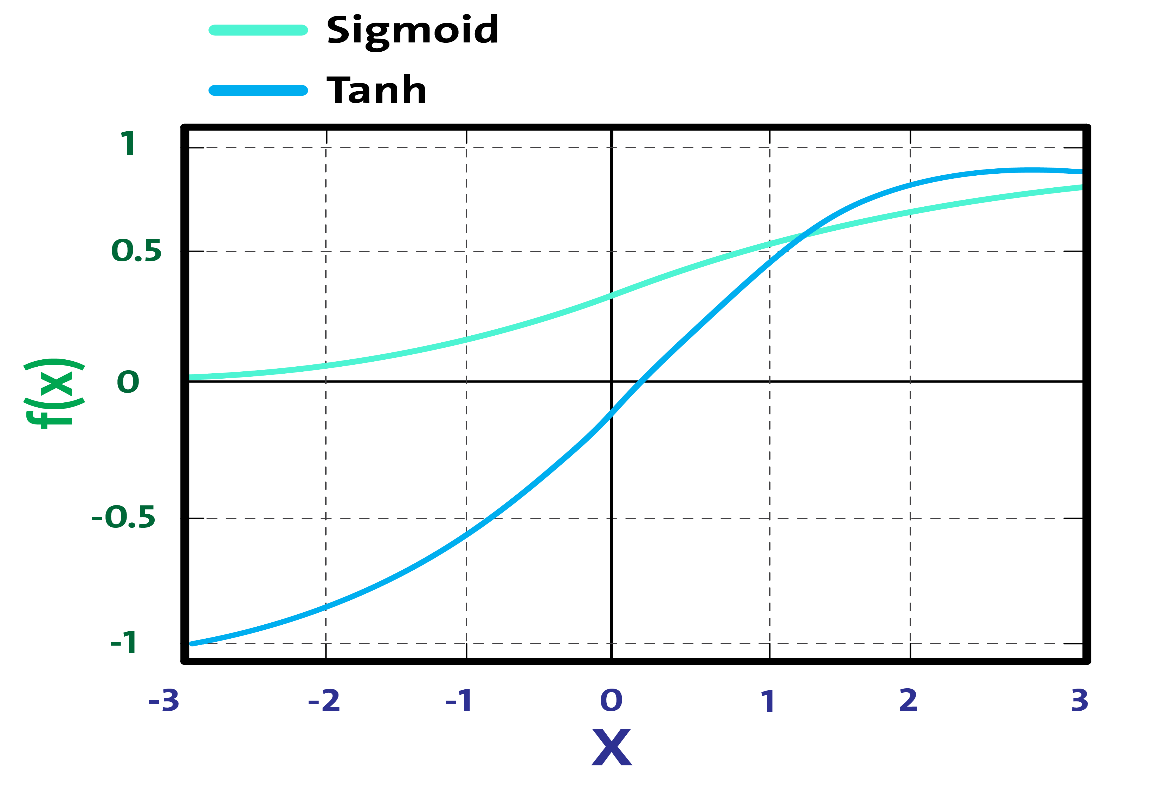Sigmoid versus tanh activation function

In general, in the last level of an feedforward neural network (FFNN), the softmax function is applied as the decision boundary. This is a common case, especially when solving a classification problem. In probability theory, the output of the softmax function is squashed as the probability distribution overKdifferent possible outcomes. Nevertheless, the softmax function is used in various multiclass classification methods, such that the network's output is distributed across classes (that is, probability distribution over the classes) having a dynamic range between -1 and 1 or 0 and 1.

### Note

For a regression problem, we do not need to use any activation function since the network generates continuous values—probabilities. However, I've seen people using the IDENTITY activation function for regression problems nowadays. We'll see this in later chapters.

To conclude, choosing proper activation functions and network weights initialization are two problems that make a network perform at its best and help to obtain good training. We'll discuss more in upcoming chapters; we will see where to use which activation function.

## Neural network architectures

There are various types of architectures in neural networks. We can categorize DL architectures into four groups: Deep Neural Networks (DNNs), Convolutional Neural Networks (CNNs), Recurrent Neural Networks (RNNs), and Emergent Architectures (EAs).

Nowadays, based on these architectures, researchers come up with so many variants of these for domain-specific use cases and research problems. The following sections of this chapter will give a brief introduction to these architectures. More detailed analysis, with examples of applications, will be the subject of later chapters of this book.

### Deep neural networks

DNNs are neural networks having complex and deeper architecture with a large number of neurons in each layer, and there are many connections. The computation in each layer transforms the representations in the subsequent layers into slightly more abstract representations. However, we will use the term DNN to refer specifically to the MLP, the Stacked Auto-Encoder (SAE), and Deep Belief Networks (DBNs).

SAEs and DBNs use AEs and Restricted Boltzmann Machines (RBMs) as building blocks of the architectures. The main difference between these and MLPs is that training is executed in two phases: unsupervised pre-training and supervised fine-tuning.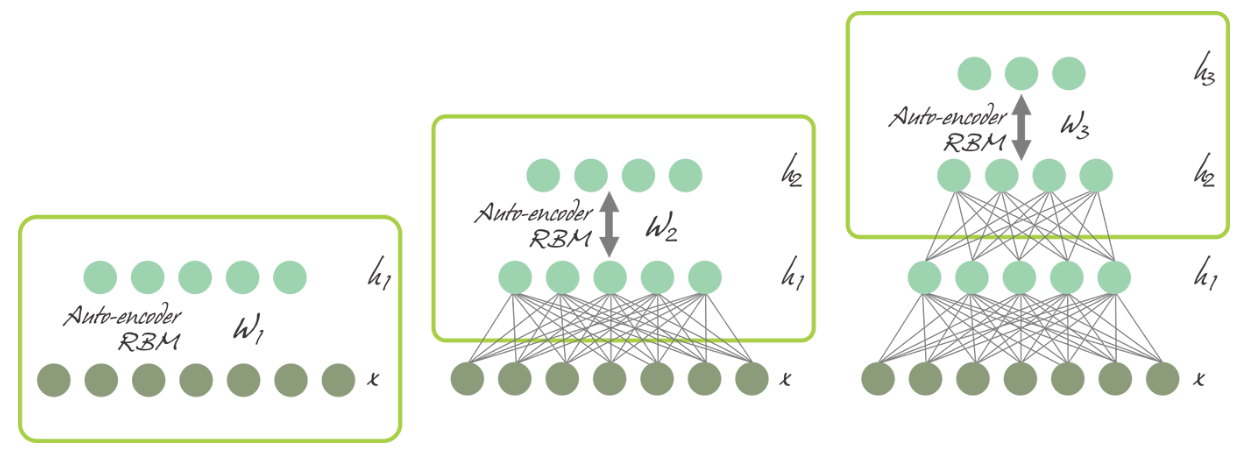SAE and DBN using AE and RBM respectively

In unsupervised pre-training, shown in the preceding diagram, the layers are stacked sequentially and trained in a layer-wise manner, like an AE or RBM using unlabeled data. Afterwards, in supervised fine-tuning, an output classifier layer is stacked and the complete neural network is optimized by retraining with labeled data.

#### Multilayer Perceptron

As discussed earlier, a single perceptron is even incapable of approximating an XOR function. To overcome this limitation, multiple perceptrons are stacked together as MLPs, where layers are connected as a directed graph. This way, the signal propagates one way, from input layer to hidden layers to output layer, as shown in the following diagram: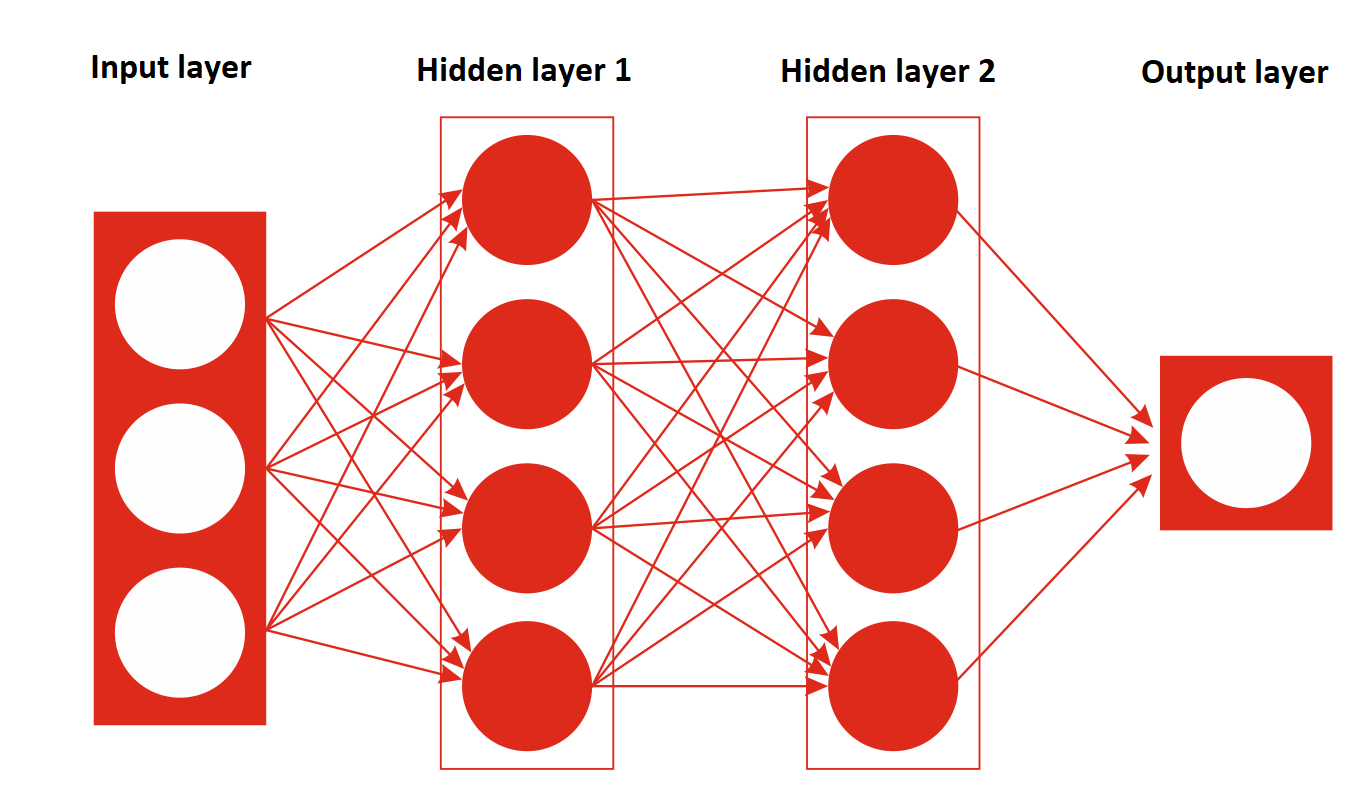An MLP architecture having an input layer, two hidden layers, and an output layer

Fundamentally, an MLP is one the most simple FFNNs having at least three layers: an input layer, a hidden layer, and an output layer. An MLP was first trained with a backpropogation algorithm in the 1980s.

#### Deep belief networks

To overcome the overfitting problem in MLPs, the DBN was proposed by Hinton et al. It uses a greedy, layer-by-layer, pre-training algorithm to initialize the network weights through probabilistic generative models.

DBNs are composed of a visible layer and multiple layers—hidden units. The top two layers have undirected, symmetric connections in between and form an associative memory, whereas lower layers receive top-down, directed connections from the preceding layer. The building blocks of a DBN are RBMs, as you can see in the following figure, where several RBMs are stacked one after another to form DBNs: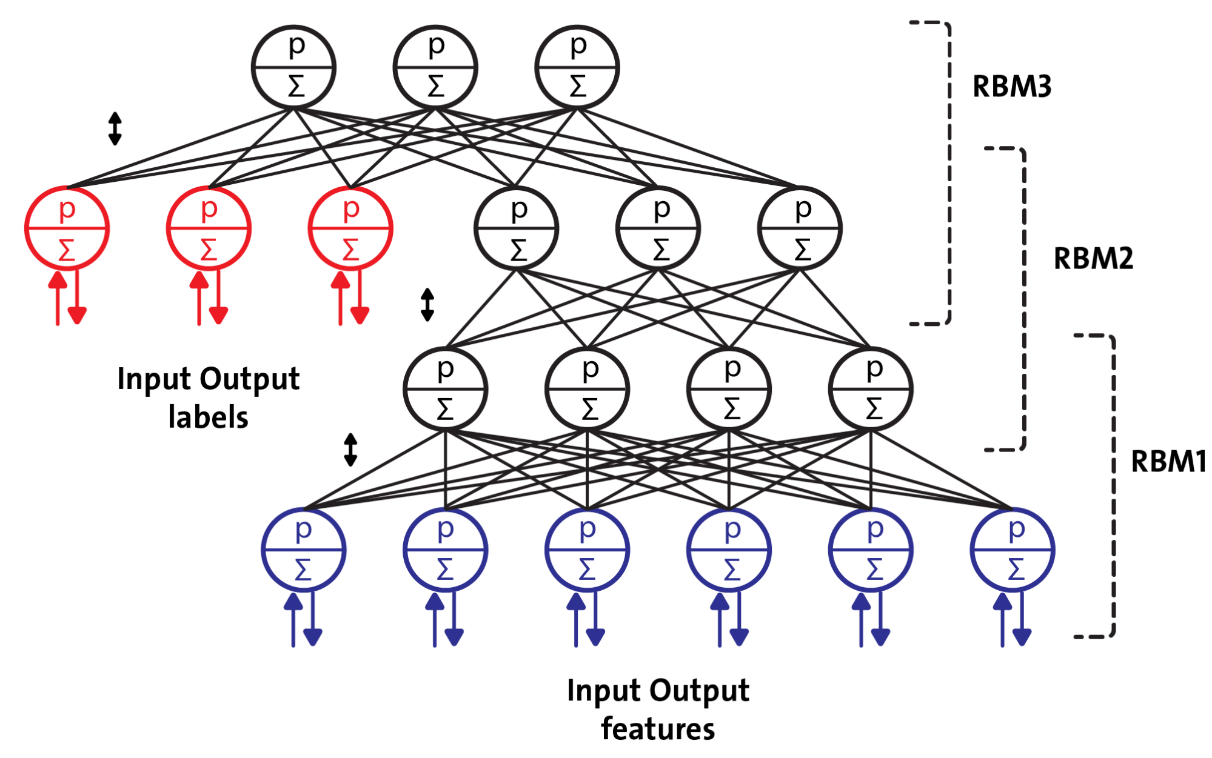A DBN configured for semi-supervised learning

A single RBM consists of two layers. The first layer is composed of visible neurons, and the second layer consists of hidden neurons. Figure 16 shows the structure of a simple RBM, where the neurons are arranged according to a symmetrical bipartite graph: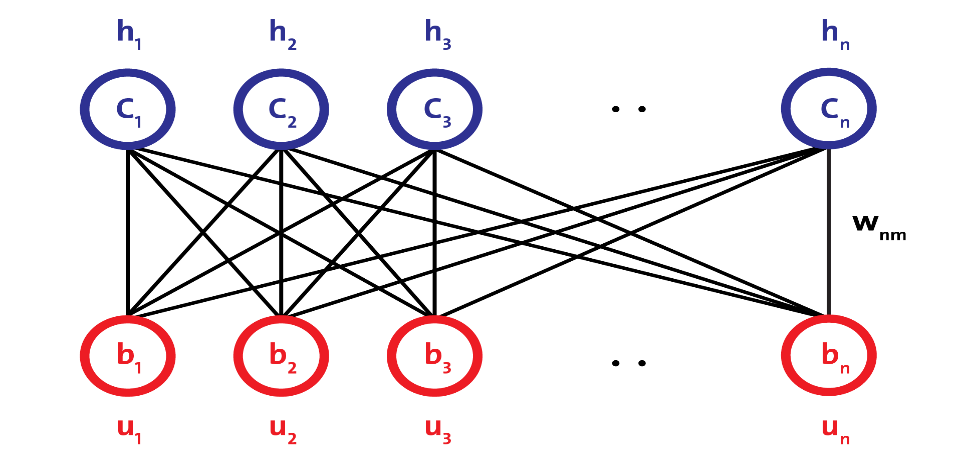RBM architecture

In DBNs, an RBM is trained first with input data, called unsupervised pre-training, and the hidden layer represents the features learned using a greedy learning approach called supervised fine-tuning. Despite numerous successes, DBNs are being replaced  by AEs.

### Autoencoders

An AE is a network with three or more layers, where the input layer and the output layer have the same number of neurons, and those intermediate (hidden layers) have a lower number of neurons. The network is trained to reproduce in the output, for each piece of input data, the same pattern of activity as in the input.

Useful applications of AEs are data denoising and dimensionality reduction for data visualization. The following diagram shows how an AE typically works. It reconstructs the received input through two phases: an encoding phase, which corresponds to a dimensional reduction for the original input, and a decoding phase, which is capable of reconstructing the original input from the encoded (compressed) representation: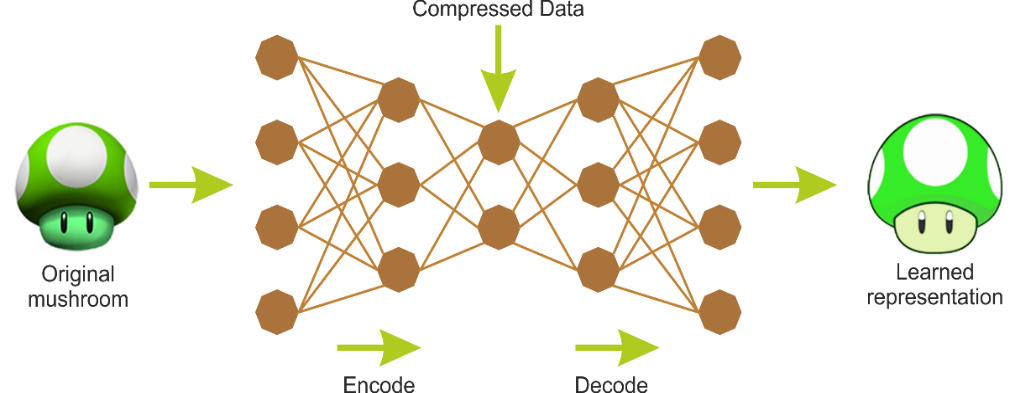Encoding and decoding phases of an AE

### Convolutional neural networks

CNNs have achieved much and wide adoption in computer vision (for example, image recognition). In CNN networks, the connection scheme that defines the convolutional layer (conv) is significantly different compared to an MLP or DBN.

Importantly, a DNN has no prior knowledge of how the pixels are organized; it does not know that nearby pixels are close. A CNN's architecture embeds this prior knowledge. Lower layers typically identify features in small areas of the image, while higher layers combine lower-level features into larger features. This works well with most natural images, giving CNNs a decisive head start over DNNs: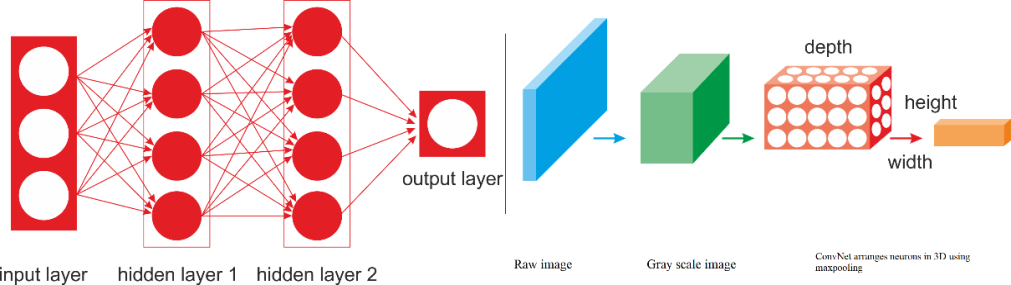A regular DNN versus a CNN

Take a close look at the preceding diagram; on the left is a regular three-layer neural network, and on the right, a CNN arranges its neurons in three dimensions (width, height, and depth). In a CNN architecture, a few convolutional layers are connected in a cascade style, where each layer is followed by a ReLU layer, then a pooling layer, then a few more convolutional layers (+ReLU), then another pooling layer, and so on.

The output from each conv layer is a set of objects called feature maps that are generated by a single kernel filter. Then the feature maps can be used to define a new input to the next layer. Each neuron in a CNN network produces an output followed by an activation threshold, which is proportional to the input and not bound. This type of layer is called a convolutional layer. The following diagram is a schematic of the architecture of a CNN used for facial recognition: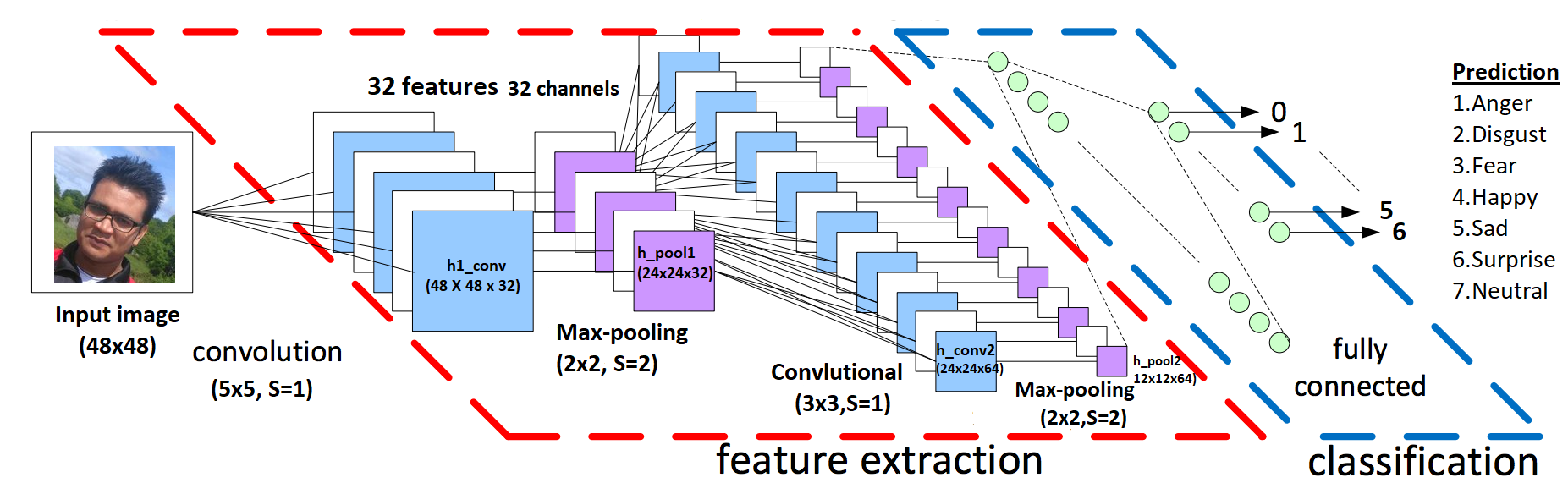A schematic architecture of a CNN used for facial recognition

### Recurrent neural networks

A recurrent neural network(RNN) is a class of artificial neural network (ANN) where connections between units form a directed cycle. RNN architecture was originally conceived by Hochreiter and Schmidhuber in 1997. RNN architectures have standard MLPs plus added loops (as shown in the following diagram), so they can exploit the powerful nonlinear mapping capabilities of the MLP; and they have some form of memory: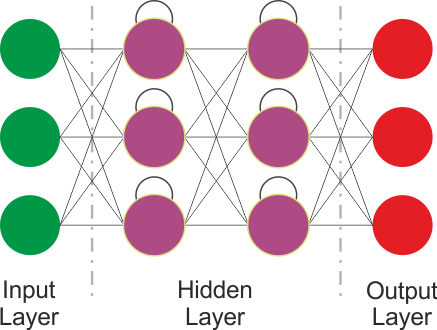RNN architecture

The preceding image shows a a very basic RNN having an input layer, 2 recurrent layers and an output layer. However, this basic RNN suffers from gradient vanishing and exploding problem and cannot model the long-term depedencies. Therefore, more advanced architectures are designed to utilize sequential information of input data with cyclic connections among building blocks such as perceptrons. These architectures include Long-Short-Term Memory (LSTM), Gated Recurrent Units (GRUs), Bidirectional-LSTM and other variants.

Consequently, LSTM and GR can overcome the drawbacks of regular RNNs: gradient vanishing/exploding problem and the long-short term dependency. We will look at these architectures in chapter 2.

### Emergent architectures

Many other emergent DL architectures have been suggested, such as Deep SpatioTemporal Neural Networks (DST-NNs), Multi-Dimensional Recurrent Neural Networks (MD-RNNs), and Convolutional AutoEncoders (CAEs).

Nevertheless, there are a few more emerging networks, such as CapsNets (which is an improved version of a CNN, designed to remove the drawbacks of regular CNNs), RNN for image recognition, and Generative Adversarial Networks (GANs) for simple image generation. Apart from these, factorization machines for personalization and deep reinforcement learning are also being used widely.

#### Residual neural networks

Since there are sometimes millions of billions of hyperparameters and other practical aspects, it's really difficult to train deeper neural networks. To overcome this limitation, Kaiming He et al. (see https://arxiv.org/abs/1512.03385v1) proposed a residual learning framework to ease the training of networks that are substantially deeper than those used previously.

They also explicitly reformulated the layers as learning residual functions with reference to the layer inputs, instead of learning unreferenced functions. This way, these residual networks are easier to optimize and can gain accuracy from considerably increased depth.

The downside is that building a network by simply stacking residual blocks inevitably limits its optimization ability. To overcome this limitation, Ke Zhang et al. also proposed using a Multilevel Residual Network (https://arxiv.org/abs/1608.02908).

GANs are deep neural net architectures that consist of two networks pitted against each other (hence the name "adversarial"). Ian Goodfellow et al. introduced GANs in a paper (see more at https://arxiv.org/abs/1406.2661v1). In GANs, the two main components are the generatorand discriminator.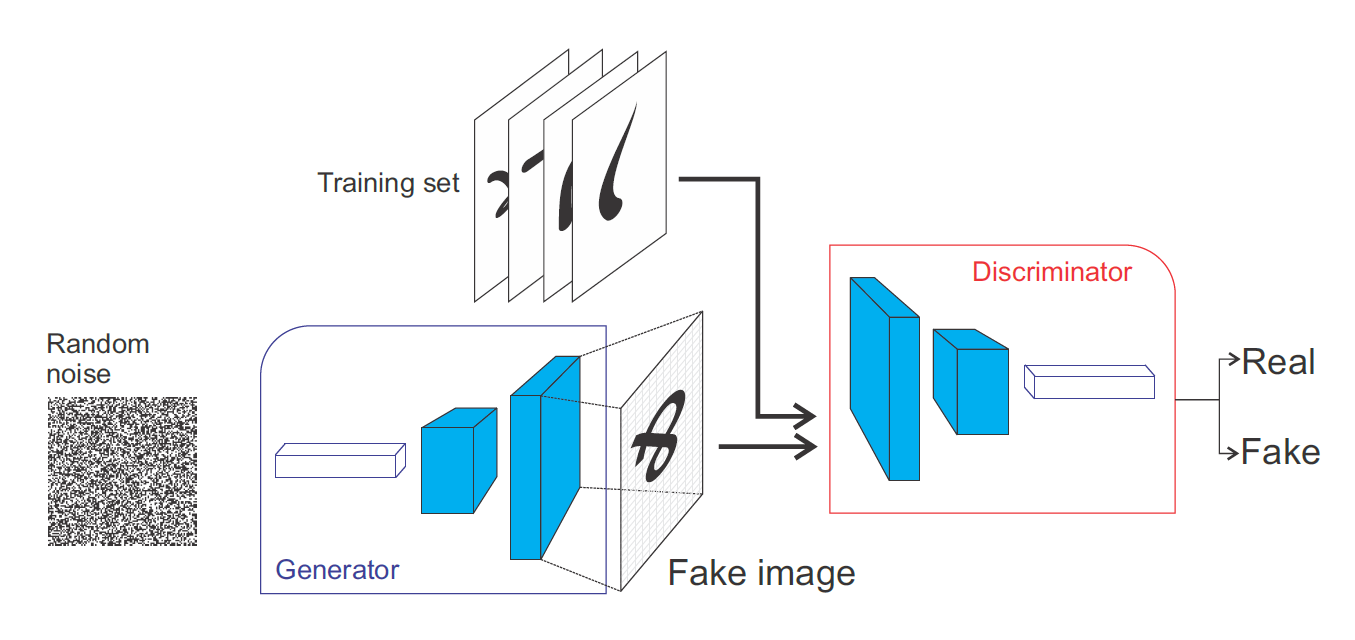Working principle of Generative Adversarial Networks (GANs)

The Generator will try to generate data samples out of a specific probability distribution, which is very similar to the actual object. The discriminator will judge whether its input is coming from the original training set or from the generator part.

#### Capsule networks

CNNs perform well at classifying images. However, if the images have rotation, tilt, or any other different orientation, then CNNs show relatively very poor performance. Even the pooling operation in CNNs cannot much help against the positional invariance.

This issue in CNNs has led us to the recent advancement of CapsNet through the paper titled Dynamic Routing Between Capsules (see more at https://arxiv.org/abs/1710.09829) by Geoffrey Hinton et al.

Unlike a regular DNN, where we keep on adding layers, in CapsNets, the idea is to add more layers inside a single layer. This way, a CapsNet is a nested set of neural layers. We'll discuss more in Chapter 11, Discussion, Current Trends, and Outlook.

## DL frameworks and cloud platforms

In this section, we'll present some of the most popular deep learning frameworks. Then we will discuss some cloud based platforms where you can deploy/run your DL applications. In short, almost all of the libraries provide the possibility of using a graphics processor to speed up the learning process, are released under an open license, and are the result of university research groups.

### Deep learning frameworks

TensorFlow is mathematical software, and an open source software library for machine intelligence. The Google Brain team developed it in 2011 and open-sourced it in 2015. The main features offered by the latest release of TensorFlow (v1.8 during the writing of this book) are faster computing, flexibility, portability, easy debugging, a unified API, transparent use of GPU computing, easy use, and extensibility. Once you have constructed your neural network model, after the necessary feature engineering, you can simply perform the training interactively using plotting or TensorBoard.

Keras is a deep learning library that sits atop TensorFlow and Theano, providing an intuitive API inspired by Torch. It is perhaps the best Python API in existence. DeepLearning4J relies on Keras as its Python API and imports models from Keras and through Keras from Theano and TensorFlow.

Theano is also a deep learning framework written in Python. It allows using GPU, which is 24x faster than a single CPU. Defining, optimizing, and evaluating complex mathematical expressions is very straightforward in Theano.

Neon is a Python-based deep learning framework developed by Nirvana. Neon has a syntax similar to Theano's high-level framework (for example, Keras). Currently, Neon is considered the fastest tool for GPU-based implementation, especially for CNNs. But its CPU-based implementation is relatively worse than most other libraries.

PyTorch is a vast ecosystem for ML that offers a large number of algorithms and functions, including for DL and for processing various types of multimedia data, with a particular focus on parallel computing. Torch is a highly portable framework supported on various platforms, including Windows, macOS, Linux, and Android.

Caffe, developed primarily by Berkeley Vision and Learning Center (BVLC), is a framework designed to stand out because of its expression, speed, and modularity.

MXNet (http://mxnet.io/) is a deep learning framework that supports many languages, such as R, Python, C++, and Julia. This is helpful because if you know any of these languages, you will not need to step out of your comfort zone at all to train your deep learning models. Its backend is written in C++ and CUDA and it is able to manage its own memory in a way similar to Theano.

The Microsoft Cognitive Toolkit (CNTK) is a unified deep learning toolkit from Microsoft Research that makes it easy to train and combine popular model types across multiple GPUs and servers. CNTK implements highly efficient CNN and RNN training for speech, image, and text data. It supports cuDNN v5.1 for GPU acceleration.

DeepLearning4J is one of the first commercial-grade, open source, distributed deep learning libraries written for Java and Scala. This also provides integrated support for Hadoop and Spark. DeepLearning4 is designed to be used in business environments on distributed GPUs and CPUs.

DeepLearning4J aims to be cutting-edge and plug-and-play, with more convention than configuration, which allows for fast prototyping for non-researchers. The following libraries can be integrated with DeepLearning4 and will make your JVM experience easier whether you are developing your ML application in Java or Scala.

ND4J is just like NumPy for JVM. It comes with some basic operations of linear algebra such as matrix creation, addition, and multiplication. ND4S, on the other hand, is a scientific computing library for linear algebra and matrix manipulation. It supports n-dimensional arrays for JVM-based languages.

To conclude, the following figure shows the last 1 year's Google trends concerning the popularity of different DL frameworks: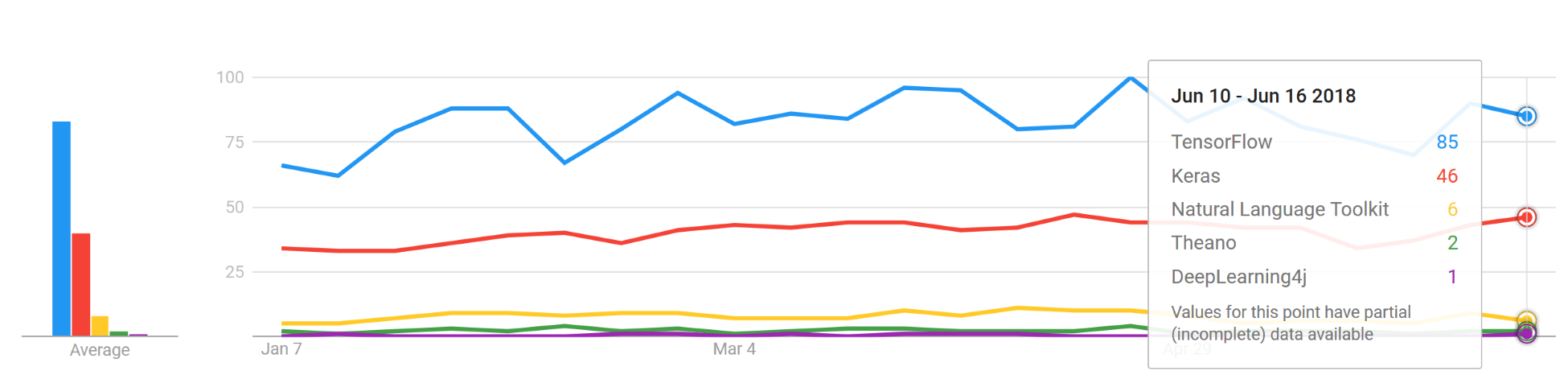The trends of different DL frameworks. TensorFlow and Keras are most dominating. Theano is losing its popularity. On the other hand, DeepLearning4J is emerging for JVM.

### Cloud-based platforms for DL

Apart from the preceding libraries, there have been some recent initiatives for deep learning on the cloud. The idea is to bring deep learning capabilities to big data with millions of billions of data points and high-dimensional data. For example, Amazon Web Services (AWS), Microsoft Azure, Google Cloud Platform, and NVIDIA GPU Cloud (NGC) all offer machine and deep learning services that are native to their public clouds.

In October 2017, AWS released deep learning Amazon Machine Images (AMIs) for Amazon Elastic Compute Cloud (EC2) P3 instances. These AMIs come pre-installed with deep learning frameworks, such as TensorFlow, Gluon, and Apache MXNet, that are optimized for the NVIDIA Volta V100 GPUs within Amazon EC2 P3 instances.

The Microsoft Cognitive Toolkit is Azure's open source, deep learning service. Similar to AWS's offering, it focuses on tools that can help developers build and deploy deep learning applications.

On the other hand, NGC empowers AI scientists and researchers with GPU-accelerated containers (see https://www.nvidia.com/en-us/data-center/gpu-cloud-computing/). NGC features containerized deep learning frameworks such as TensorFlow, PyTorch, MXNet, and more that are tuned, tested, and certified by NVIDIA to run on the latest NVIDIA GPUs.

Now that we have a minimum of knowledge about available DL libraries, frameworks, and cloud-based platforms for running and deploying our DL applications, we can dive into coding. First, we will start by solving the famous Titanic survival prediction problem. However, we won't use the previously listed frameworks; we will be using the Apache Spark ML library. Since we will be using Spark along with other DL libraries, knowing a little bit of Spark would help us grasp things in the upcoming chapters.

## Deep learning from a disaster – Titanic survival prediction

In this section, we are going to solve the famous Titanic survival prediction problem available on Kaggle (see https://www.kaggle.com/c/titanic/data). The task is to complete the analysis of what sorts of people are likely to survive using an ML algorithm.

### Problem description

Before diving into the coding, let's see a short description of the problem. This paragraph is directly quoted from the Kaggle Titanic survival prediction page:

"The sinking of the RMS Titanic is one of the most infamous shipwrecks in history. On April 15, 1912, during her maiden voyage, the Titanic sank after colliding with an iceberg, killing 1502 out of 2224 passengers and crew. This sensational tragedy shocked the international community and led to better safety regulations for ships. One of the reasons that the shipwreck led to such loss of life was that there were not enough lifeboats for the passengers and crew. Although there was some element of luck involved in surviving the sinking, some groups of people were more likely to survive than others, such as women, children, and the upper class. In this challenge, we ask you to complete the analysis of what sorts of people were likely to survive. In particular, we ask you to apply the tools of machine learning to predict which passengers survived the tragedy."

Now, before going even deeper, we need to know about the data of the passengers traveling on the Titanic during the disaster so that we can develop a predictive model that can be used for survival analysis. The dataset can be downloaded from https://github.com/rezacsedu/TitanicSurvivalPredictionDataset. There are two `.csv` files:

• The training set (`train.csv`): Can be used to build your ML models. This file also includes labels as the ground truth for each passenger for the training set.
• The test set (`test.csv`): Can be used to see how well your model performs on unseen data. However, for the test set, we do not provide the ground truth for each passenger.

In short, for each passenger in the test set, we have to use the trained model to predict whether they'll survive the sinking of the Titanic. Table 1 shows the metadata of the training set:

 Variable Definition `survival` Two labels:0 = No1 = Yes `pclass` This is a proxy for the Socioeconomic Status (SES) of a passenger and is categorized as upper, middle, and lower. In particular, 1 = 1st, 2 = 2nd, 3 = 3rd. `sex` Male or female. `Age` Age in years. `sibsp` This signifies family relations as follows:Sibling = brother, sister, stepbrother, stepsisterSpouse = husband, wife (mistresses and fiancés were ignored) `parch` In the dataset, family relations are defined as follows:Parent = mother, fatherChild = daughter, son, stepdaughter, stepsonSome children traveled only with a nanny, therefore parch=0 for them. `ticket` Ticket number. `fare` Passenger ticket fare. cabin Cabin number. `embarked` Three ports:C = CherbourgQ = QueenstownS = Southampton

Now the question would be: using this labeled data, can we draw some straightforward conclusions? Say that being a woman, being in first class, and being a child were all factors that could boost a passenger's chances of survival during this disaster.

To solve this problem, we can start from the basic MLP, which is one of the oldest deep learning algorithms. For this, we use the Spark-based `MultilayerPerceptronClassifier`. At this point, you might be wondering why I am talking about Spark since it is not a DL library. However, Spark has an MLP implementation, which would be enough to serve our objective.

Then from the next chapter, we'll gradually start using more robust DNN by using DeepLearning4J, a JVM-based framework for developing deep learning applications. So let's see how to configure our Spark environment.

### Configuring the programming environment

I am assuming that Java is already installed on your machine and the `JAVA_HOME` is set too. Also, I'm assuming that your IDE has the Maven plugin installed. If so, then just create a Maven project and add the project properties as follows:

```<properties>
<project.build.sourceEncoding>UTF-8</project.build.sourceEncoding>
<java.version>1.8</java.version>
<jdk.version>1.8</jdk.version>
<spark.version>2.3.0</spark.version>
</properties>```

In the preceding tag, I specified Spark (that is, 2.3.0), but you can adjust it. Then add the following dependencies in the `pom.xml` file:

```<dependencies>
<dependency>
<groupId>org.apache.spark</groupId>
<artifactId>spark-core_2.11</artifactId>
<version>\${spark.version}</version>
</dependency>
<dependency>
<groupId>org.apache.spark</groupId>
<artifactId>spark-sql_2.11</artifactId>
<version>\${spark.version}</version>
</dependency>
<dependency>
<groupId>org.apache.spark</groupId>
<artifactId>spark-mllib_2.11</artifactId>
<version>\${spark.version}</version>
</dependency>
<dependency>
<groupId>org.apache.spark</groupId>
<artifactId>spark-graphx_2.11</artifactId>
<version>\${spark.version}</version>
</dependency>
<dependency>
<groupId>org.apache.spark</groupId>
<artifactId>spark-yarn_2.11</artifactId>
<version>\${spark.version}</version>
</dependency>
<dependency>
<groupId>org.apache.spark</groupId>
<artifactId>spark-network-shuffle_2.11</artifactId>
<version>\${spark.version}</version>
</dependency>
<dependency>
<groupId>org.apache.spark</groupId>
<artifactId>spark-streaming-flume_2.11</artifactId>
<version>\${spark.version}</version>
</dependency>
<dependency>
<groupId>com.databricks</groupId>
<artifactId>spark-csv_2.11</artifactId>
<version>1.3.0</version>
</dependency>
</dependencies>```

Then if everything goes smoothly, all the JAR files will be downloaded in the project home as Maven dependencies. Alright! Then we can start writing the code.

### Feature engineering and input dataset preparation

In this sub-section, we will see some basic feature engineering and dataset preparation that can be fed into the MLP classifier. So let's start by creating `SparkSession`, which is the gateway to access Spark:

```SparkSession spark = SparkSession
.builder()
.master("local[*]")
.config("spark.sql.warehouse.dir", "/tmp/spark")
.appName("SurvivalPredictionMLP")
.getOrCreate();```

Then let's read the training set and see a glimpse of it:

```Dataset<Row> df = spark.sqlContext()
.format("com.databricks.spark.csv")
.option("inferSchema", "true")
df.show();```

A snapshot of the dataset can be seen as follows: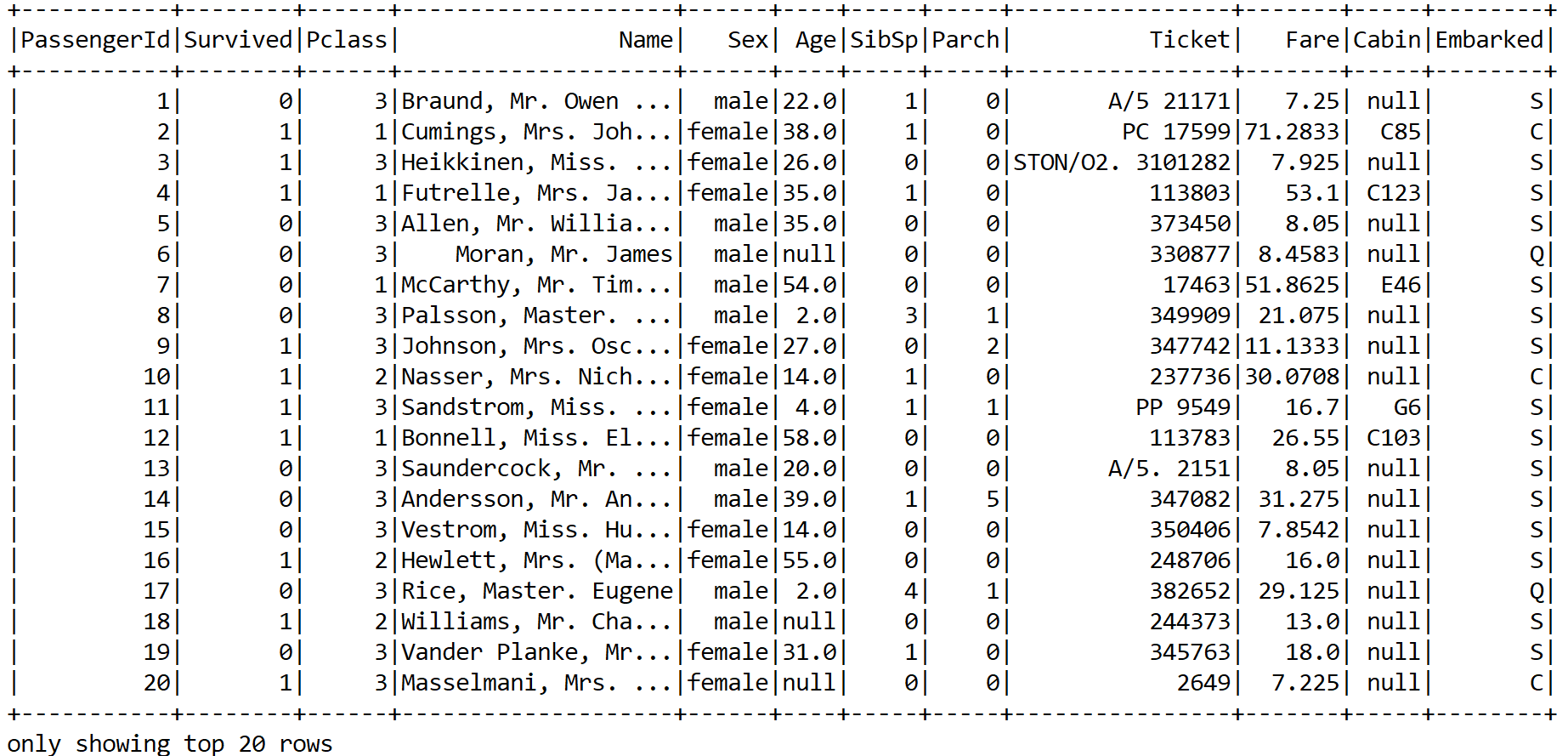A snapshot of the Titanic survival dataset

Now we can see that the training set has both categorical as well as numerical features. In addition, some features are not important, such as `PassengerID`, `Ticket`, and so on. The same also applies to the `Name` feature unless we manually create some features based on the title. However, let's keep it simple. Nevertheless, some columns contain null values. Therefore, lots of consideration and cleaning are required.

I ignore the `PassengerId`, `Name`, and `Ticket` columns. Apart from these, the `Sex` column is categorical, so I've encoded the passengers based on `male` and `female`. Then the `Embarked` column is encoded too. We can encode `S` as `0`, `C` as `1`, and `Q` as `2`.

For this also, we can write user-defined-functions (also known as UDFs) called `normSex` and `normEmbarked` for `Sex` and `Embarked`, respectively. Let's see their signatures:

```privatestatic UDF1<String,Option<Integer>> normEmbarked=(String d) -> {
if (null == d)
return Option.apply(null);
else {
if (d.equals("S"))
return Some.apply(0);
elseif (d.equals("C"))
return Some.apply(1);
else
return Some.apply(2);
}
};```

Therefore, this UDF takes a `String` type and encodes as an integer. Now the `normSex` UDF also works similarly:

```privatestatic UDF1<String, Option<Integer>> normSex = (String d) -> {
if (null == d)
return Option.apply(null);
else {
if (d.equals("male"))
return Some.apply(0);
else
return Some.apply(1);
}
};```

So we can now select only useful columns but for the `Sex` and `Embarked` columns with the aforementioned UDFs:

```Dataset<Row> projection = df.select(
col("Survived"),
col("Fare"),
callUDF("normSex", col("Sex")).alias("Sex"),
col("Age"),
col("Pclass"),
col("Parch"),
col("SibSp"),
callUDF("normEmbarked",
col("Embarked")).alias("Embarked"));
projectin.show();```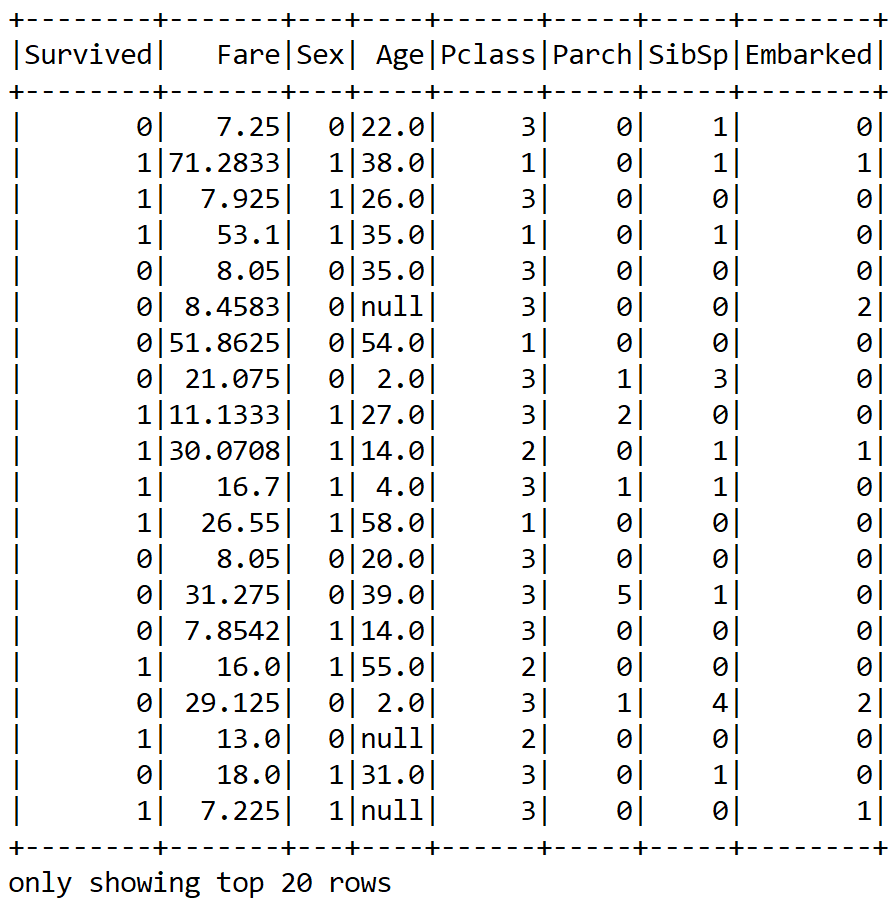Now we have been able to convert a categorical column into a numeric; however, as we can see, there are still null values. Therefore, what can we do? We can either drop the `null` values altogether or apply some `null` imputing techniques with the mean value of those particular columns. I believe the second approach is better.

Now, again for this null imputation, we can write UDFs too. However, for that we need to know some statistics about those numerical columns. Unfortunately, we cannot perform the summary statistics on DataFrame. Therefore, we have to convert the DataFrame into `JavaRDD<Vector>`. Well, we also ignore the `null` entries for calculating this:

```JavaRDD<Vector> statsDf =projection.rdd().toJavaRDD().map(row -> Vectors.dense( row.<Double>getAs("Fare"),
row.isNullAt(3) ? 0d : row.Double>getAs("Age")
));```

Now let's compute the multivariate statistical `summary`. The `summary` statistical will be further used to calculate the `meanAge` and `meanFare` for the corresponding missing entries for these two features:

```MultivariateStatisticalSummary summary = Statistics.colStats(statsRDD.rdd());
double meanFare = summary.mean().apply(0);
double meanAge = summary.mean().apply(1); ```

Now let's create two more UDFs for the null imputation on the `Age` and `Fare` columns:

```UDF1<String, Option<Double>> normFare = (String d) -> {
if (null == d) {
return Some.apply(meanFare);
}
else
return Some.apply(Double.parseDouble(d));
};```

Therefore, we have defined a UDF, which fills in the `meanFare` values if the data has no entry. Now let's create another UDF for the `Age` column:

```UDF1<String, Option<Double>> normAge = (String d) -> {
if (null == d)
return Some.apply(meanAge);
else
return Some.apply(Double.parseDouble(d));
};```

Now we need to register the UDFs as follows:

```spark.sqlContext().udf().register("normFare", normFare, DataTypes.DoubleType);
spark.sqlContext().udf().register("normAge", normAge, DataTypes.DoubleType);```

Therefore, let's apply the preceding UDFs for `null` imputation:

```Dataset<Row> finalDF = projection.select(
col("Survived"),
callUDF("normFare",
col("Fare").cast("string")).alias("Fare"),
col("Sex"),
callUDF("normAge",
col("Age").cast("string")).alias("Age"),
col("Pclass"),
col("Parch"),
col("SibSp"),
col("Embarked"));
finalDF.show();```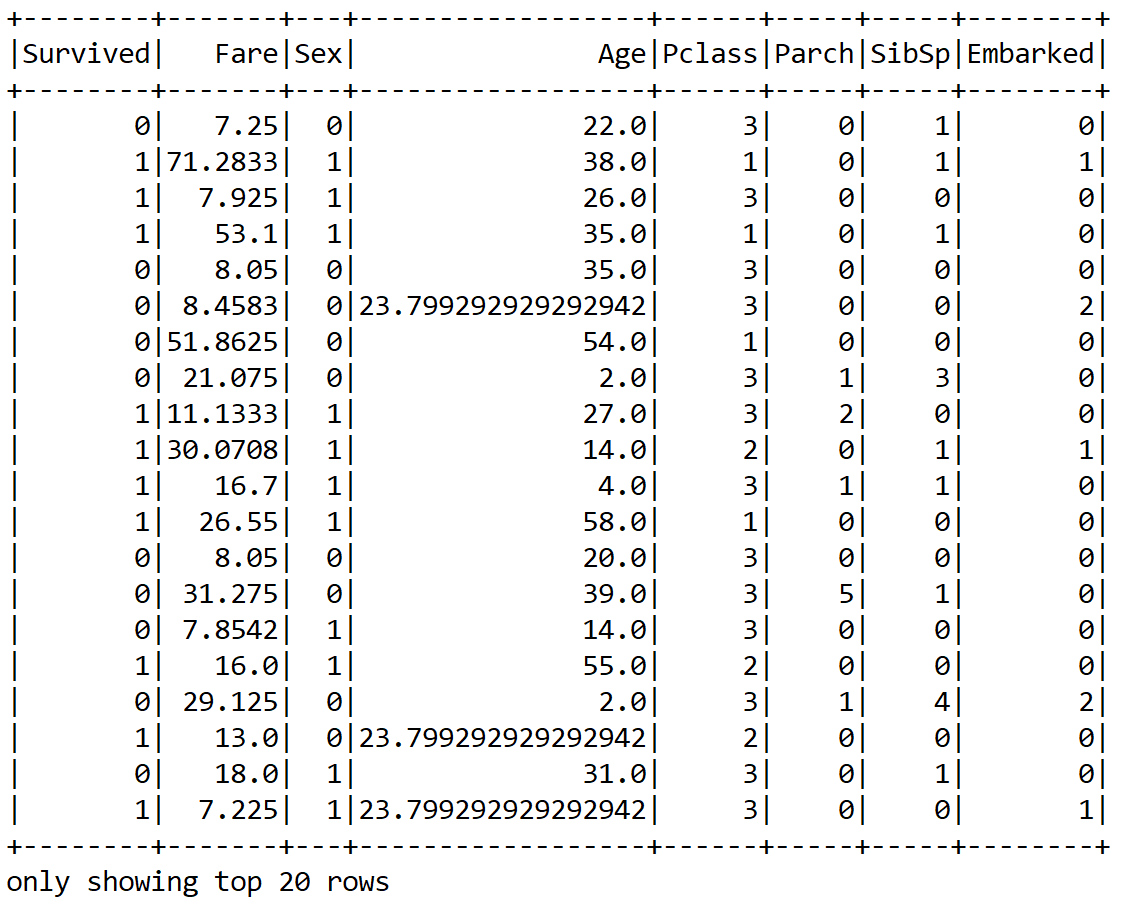Great! We now can see that the `null` values are replaced with the mean value for the `Age` and `Fare` columns. However, still the numeric values are not scaled. Therefore, it would be a better idea to scale them. However, for that, we need to compute the mean and variance and then store them as a model to be used for later scaling:

```Vector stddev = Vectors.dense(Math.sqrt(summary.variance().apply(0)), Math.sqrt(summary.variance().apply(1)));

Vector mean = Vectors.dense(summary.mean().apply(0), summary.mean().apply(1));
StandardScalerModel scaler = new StandardScalerModel(stddev, mean);```

Then we need an encoder for the numeric values (that is, `Integer`; either `BINARY` or `Double`):

```Encoder<Integer> integerEncoder = Encoders.INT();
Encoder<Double> doubleEncoder = Encoders.DOUBLE();
Encoders.BINARY();

Encoder<Vector> vectorEncoder = Encoders.kryo(Vector.class);
Encoders.tuple(integerEncoder, vectorEncoder);
Encoders.tuple(doubleEncoder, vectorEncoder);```

Then we can create a `VectorPair` consisting of the label (that is, `Survived`) and the features. Here the encoding is, basically, creating a scaled feature vector:

```JavaRDD<VectorPair> scaledRDD = trainingDF.toJavaRDD().map(row -> {
VectorPair vectorPair = new VectorPair();
vectorPair.setLable(new
Double(row.<Integer> getAs("Survived")));

vectorPair.setFeatures(Util.getScaledVector(
row.<Double>getAs("Fare"),
row.<Double>getAs("Age"),
row.<Integer>getAs("Pclass"),
row.<Integer>getAs("Sex"),
row.isNullAt(7) ? 0d :
row.<Integer>getAs("Embarked"),
scaler));
return vectorPair;
});```

In the preceding code block, the `getScaledVector()` method does perform the scaling operation. The signature of this method can be seen as follows:

```publicstatic org.apache.spark.mllib.linalg.Vector getScaledVector(double fare,
double age, double pclass,  double sex, double embarked, StandardScalerModel scaler) {
org.apache.spark.mllib.linalg.Vector scaledContinous = scaler.transform(Vectors.dense(fare, age));
Tuple3<Double, Double, Double> pclassFlat = flattenPclass(pclass);
Tuple3<Double, Double, Double> embarkedFlat = flattenEmbarked(embarked);
Tuple2<Double, Double> sexFlat = flattenSex(sex);

return Vectors.dense(
scaledContinous.apply(0),
scaledContinous.apply(1),
sexFlat._1(),
sexFlat._2(),
pclassFlat._1(),
pclassFlat._2(),
pclassFlat._3(),
embarkedFlat._1(),
embarkedFlat._2(),
embarkedFlat._3());
}```

Since we planned to use a Spark ML-based classifier (that is, an MLP implementation), we need to convert this RDD of the vector to an ML vector:

`Dataset<Row> scaledDF = spark.createDataFrame(scaledRDD, VectorPair.class);`

Finally, let's see how the resulting DataFrame looks:

`scaledDF.show();`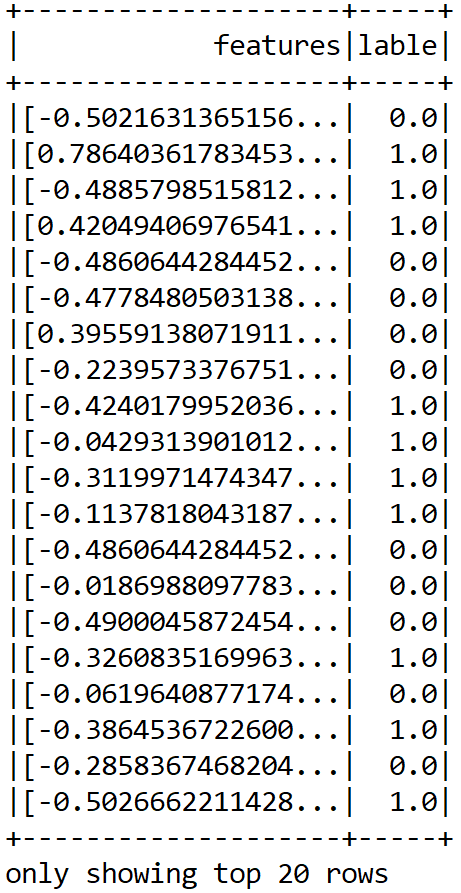Up to this point, we have been able to prepare our features. Still, this is an MLlib-based vector, so we need to further convert this into an ML vector:

`Dataset<Row> scaledData2 = MLUtils.convertVectorColumnsToML(scaledDF);`

Fantastic! Now were' almost done preparing a training set that can be consumed by the MLP classifier. Since we also need to evaluate the model's performance, we can randomly split the training data for the training and test sets. Let's allocate 80% for training and 20% for testing. These will be used to train the model and evaluate the model, respectively:

```Dataset<Row> data = scaledData2.toDF("features", "label");
Dataset<Row>[] datasets = data.randomSplit(newdouble[]{0.80, 0.20}, 12345L);

Dataset<Row> trainingData = datasets;
Dataset<Row> validationData = datasets;```

Alright. Now that we have the training set, we can perform training on an MLP model.

### Training MLP classifier

In Spark, an MLP is a classifier that consists of multiple layers. Each layer is fully connected to the next layer in the network. Nodes in the input layer represent the input data, whereas other nodes map inputs to outputs by a linear combination of the inputs with the node’s weights and biases and by applying an activation function.

So let's create the layers for the MLP classifier. For this example, let's make a shallow network considering the fact that our dataset is not that highly dimensional.

Let's assume that only 18 neurons in the first hidden layer and `8` neurons in the second hidden layer would be sufficient. Note that the input layer has `10` inputs, so we set `10` neurons and `2` neurons in the output layers since our MLP will predict only `2` classes. One thing is very important—the number of inputs has to be equal to the size of the feature vectors and the number of outputs has to be equal to the total number of labels:

`int[] layers = newint[] {10, 8, 16, 2};`

Then we instantiate the model with the trainer and set its parameters:

```MultilayerPerceptronClassifier mlp = new MultilayerPerceptronClassifier()
.setLayers(layers)
.setBlockSize(128)
.setSeed(1234L)
.setTol(1E-8)
.setMaxIter(1000);```

So, as you can understand, the preceding `MultilayerPerceptronClassifier()` is the classifier trainer based on the MLP. Each layer has a sigmoid activation function except the output layer, which has the softmax activation. Note that Spark-based MLP implementation supports only minibatch GD and LBFGS optimizers.

In short, we cannot use other activation functions such as ReLU or tanh in the hidden layers. Apart from this, other advanced optimizers are also not supported, nor are batch normalization and so on. This is a serious constraint of this implementation. In the next chapter, we will try to overcome this with DL4J.

We have also set the convergence tolerance of iterations as a very small value so that it will lead to higher accuracy with the cost of more iterations. We set the block size for stacking input data in matrices to speed up the computation.

### Note

If the size of the training set is large, then the data is stacked within partitions. If the block size is more than the remaining data in a partition, then it is adjusted to the size of this data. The recommended size is between 10 and 1,000, but the default block size is 128.

Finally, we plan to iterate the training 1,000 times. So let's start training the model using the training set:

`MultilayerPerceptronClassificationModel model = mlp.fit(trainingData);`

### Evaluating the MLP classifier

When the training is completed, we compute the prediction on the test set to evaluate the robustness of the model:

`Dataset<Row> predictions = model.transform(validationData);`

Now, how about seeing some sample predictions? Let's observe both the true labels and the predicted labels:

`predictions.show();`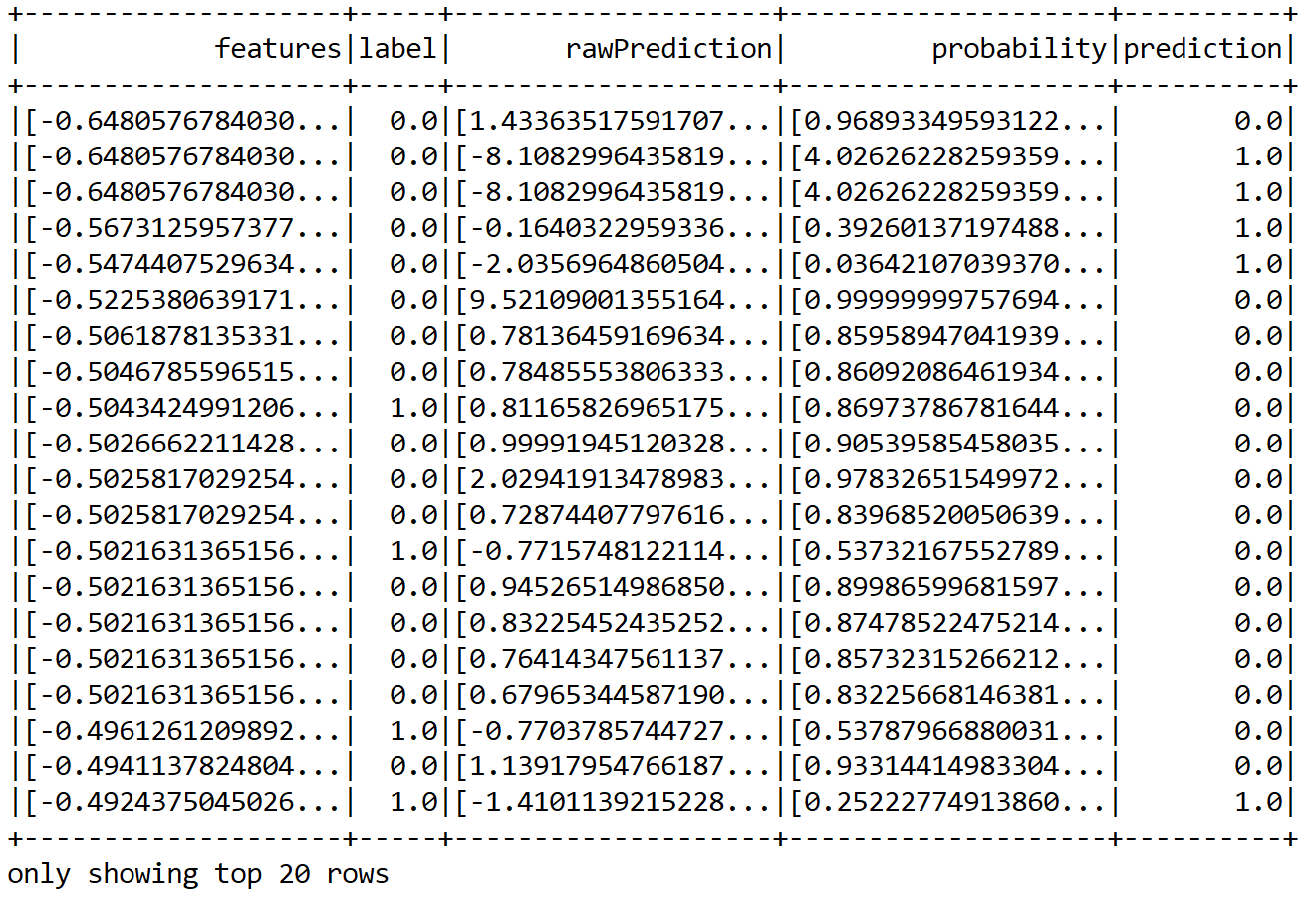We can see that some predictions are correct but some of them are wrong too. Nevertheless, in this way, it is difficult to guess the performance. Therefore, we can compute performance metrics such as precision, recall, and f1 measure:

```MulticlassClassificationEvaluator evaluator = new MulticlassClassificationEvaluator()
.setLabelCol("label")
.setPredictionCol("prediction");

MulticlassClassificationEvaluator evaluator1 = evaluator.setMetricName("accuracy");
MulticlassClassificationEvaluator evaluator2 = evaluator.setMetricName("weightedPrecision");
MulticlassClassificationEvaluator evaluator3 = evaluator.setMetricName("weightedRecall");
MulticlassClassificationEvaluator evaluator4 = evaluator.setMetricName("f1");```

Now let's compute the classification's `accuracy`, `precision`, `recall`, `f1` measure, and error on test data:

```double accuracy = evaluator1.evaluate(predictions);
double precision = evaluator2.evaluate(predictions);
double recall = evaluator3.evaluate(predictions);
double f1 = evaluator4.evaluate(predictions);

// Print the performance metrics
System.out.println("Accuracy = " + accuracy);
System.out.println("Precision = " + precision);
System.out.println("Recall = " + recall);
System.out.println("F1 = " + f1);

System.out.println("Test Error = " + (1 - accuracy));```
``````>>>
``````Accuracy = 0.7796476846282568
Precision = 0.7796476846282568
Recall = 0.7796476846282568
F1 = 0.7796476846282568
Test Error = 0.22035231537174316``````

Well done! We have been able to achieve a fair accuracy rate, that is, 78%. Still we can improve the with additional feature engineering. More tips will be given in the next section! Now, before concluding this chapter, let's try to utilize the trained model to get the prediction on the test set. First, we read the test set and create the DataFrame:

`Dataset<Row> testDF = Util.getTestDF();`

Nevertheless, even if you see the test set, it has some null values. So let's do null imputation on the `Age` and `Fare` columns. If you don't prefer using UDF, you can create a MAP where you include your imputing plan:

```Map<String, Object> m = new HashMap<String, Object>();
m.put("Age", meanAge);
m.put("Fare", meanFare);

Dataset<Row> testDF2 = testDF.na().fill(m);```

Then again, we create an RDD of `vectorPair` consisting of features and labels (target column):

```JavaRDD<VectorPair> testRDD = testDF2.javaRDD().map(row -> {
VectorPair vectorPair = new VectorPair();
vectorPair.setLable(row.<Integer>getAs("PassengerId"));
vectorPair.setFeatures(Util.getScaledVector(
row.<Double>getAs("Fare"),
row.<Double>getAs("Age"),
row.<Integer>getAs("Pclass"),
row.<Integer>getAs("Sex"),
row.<Integer>getAs("Embarked"),
scaler));
return vectorPair;
});```

Then we create a Spark DataFrame:

`Dataset<Row> scaledTestDF = spark.createDataFrame(testRDD, VectorPair.class);`

Finally, let's convert the MLib vectors to ML based vectors:

`Dataset<Row> finalTestDF = MLUtils.convertVectorColumnsToML(scaledTestDF).toDF("features", "PassengerId");`

Now, let's perform the model inferencing, that is, create a prediction for the `PassengerId` column and show the sample `prediction`:

```Dataset<Row> resultDF = model.transform(finalTestDF).select("PassengerId", "prediction");
resultDF.show();```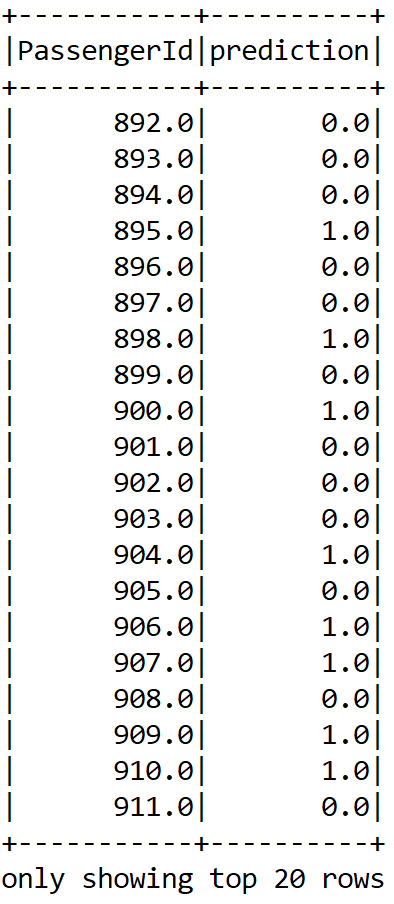Finally, let's write the result in a CSV file:

`resultDF.write().format("com.databricks.spark.csv").option("header", true).save("result/result.csv");`

Now that we have solved the Titanic survival prediction problem with an acceptable level of accuracy, there are other practical aspects of this problem and of overall deep learning phenomena that need to be considered too. In this section, we will see some frequently asked questions that might be already in your mind. Answers to these questions can be found in Appendix A.

1. Draw an ANN using the original artificial neurons that compute the XOR operation: AB. Describe this problem formally as a classification problem. Why can't simple neurons solve this problem? How does an MLP solve this problem by stacking multiple perceptrons?
2. We have briefly seen the history of ANNs. What are the most significant milestones in the era of deep learning? Can we explain the timeline in a single figure?
3. Can I use another deep learning framework for solving this Titanic survival prediction problem more flexibly?
4. Can I use `Name` as a feature to be used in the MLP in the code?
5. I understand the number of neurons in the input and output layers. But how many neurons should I set for the hidden layers?
6. Can't we improve the predictive accuracy by the cross-validation and grid search technique?

## Summary

In this chapter, we introduced some fundamental themes of DL. We started our journey with a basic but comprehensive introduction to ML. Then we gradually moved on to DL and different neural architectures. Then we got a brief overview of the most important DL frameworks. Finally, we saw some frequently asked questions related to deep learning and the Titanic survival prediction problem.

In the next chapter, we'll begin our journey into DL by solving the Titanic survival prediction problem using MLP. Then'll we start developing an end-to-end project for cancer type classification using a recurrent LSTM network. A very-high-dimensional gene expression dataset will be used for training and evaluating the model.

Answer to question 1: There are many ways to solve this problem:

1. AB= (A ∨ ¬ B)∨ (¬ A ∧ B)
2. B = (A ∨ B) ∧ ¬(A ∨ B)
3. B = (A ∨ B) ∧ (¬ A ∨ ∧ B), and so on

If we go with the first approach, the resulting ANNs would look like this: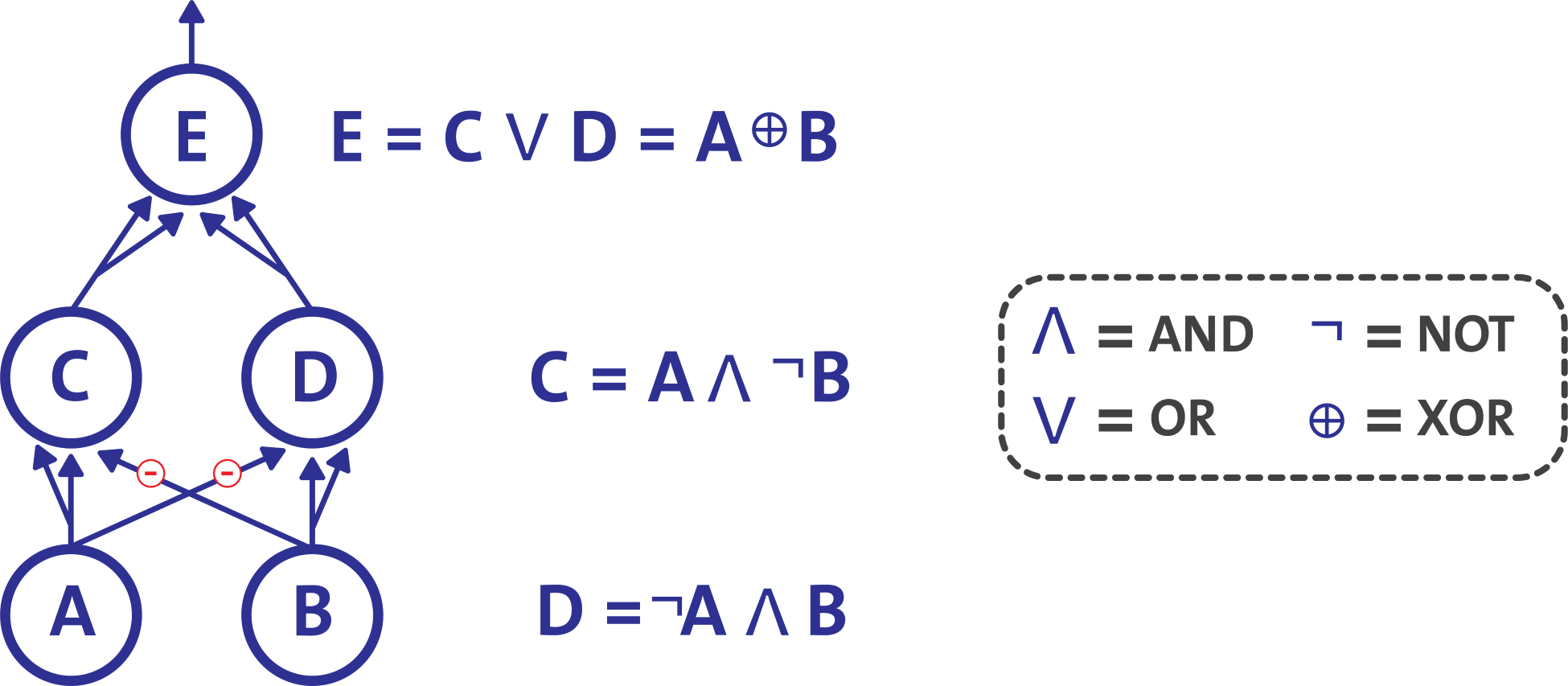Now from computer science literature, we know that only two input combinations and one output are associated with the XOR operation. With inputs (0, 0) or (1, 1) the network outputs 0; and with inputs (0, 1) or (1, 0), it outputs 1. So we can formally represent the preceding truth table as follows:

 X0 X1 Y 0 0 0 0 1 1 1 0 1 1 1 0

Here, each pattern is classified into one of two classes that can be separated by a single line L. They are known as linearly separable patterns, as represented here: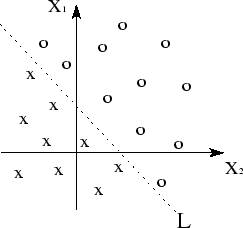Answer to question 2: The most significant progress in ANN and DL can be described in the following timeline. We have already seen how artificial neurons and perceptrons provided the base in 1943s and 1958s respectively. Then, XOR was formulated as a linearly non-separable problem in 1969 by Minsky et al. But later in 1974, Werbos et al. demonstrated the backpropagation algorithm for training the perceptron in 1974.

However, the most significant advancement happened in the 1980s, when John Hopfield et al. proposed the Hopfield Network in 1982. Then, Hinton, one of the godfathers of neural networks and deep learning, and his team proposed the Boltzmann machine in 1985. However, probably one of the most significant advances happened in 1986, when Hinton et al. successfully trained the MLP, and Jordan et. al. proposed RNNs. In the same year, Smolensky et al. also proposed an improved version of the RBM.

In the 1990s, the most significant year was 1997. Lecun et al. proposed LeNet in 1990, and Jordan et al. proposed RNN in 1997. In the same year, Schuster et al. proposed an improved version of LSTM and an improved version of the original RNN, called bidirectional RNN.

Despite significant advances in computing, from 1997 to 2005, we hadn't experienced much advancement, until Hinton struck again in 2006. He and his team proposed a DBN by stacking multiple RBMs. Then in 2012, again Hinton invented dropout, which significantly improved regularization and overfitting in a DNN.

After that, Ian Goodfellow et al. introduced GANs, a significant milestone in image recognition. In 2017, Hinton proposed CapsNets to overcome the limitations of regular CNNs—so far one of the most significant milestones.

Answer to question 3: Yes, you can use other deep learning frameworks described in the Deep learning frameworks section. However, since this book is about using Java for deep learning, I would suggest going for DeepLearning4J. We will see how flexibly we can create networks by stacking input, hidden, and output layers using DeepLearning4J in the next chapter.

Answer to question 4: Yes, you can, since the passenger's name containing a different title (for example, Mr., Mrs., Miss, Master, and so on) could be significant too. For example, we can imagine that being a woman (that is, Mrs.) and being a junior (for example, Master.) could give a higher chance of survival.

Even, after watching the famous movie Titanic (1997), we can imagine that being in a relationship, a girl might have a good chance of survival since his boyfriend would try to save her! Anyway, this is just for imagination, so do not take it seriously. Now, we can write a user-defined function to encode this using Apache Spark. Let's take a look at the following UDF in Java:

```private staticfinal UDF1<String, Option<String>> getTitle = (String name) ->      {
if(name.contains("Mr.")) { // If it has Mr.
return Some.apply("Mr.");
} else if(name.contains("Mrs.")) { // Or if has Mrs.
return Some.apply("Mrs.");
} else if(name.contains("Miss.")) { // Or if has Miss.
return Some.apply("Miss.");
} else if(name.contains("Master.")) { // Or if has Master.
return Some.apply("Master.");
} else{ // Not any.
return Some.apply("Untitled");
}
};```

Next, we can register the UDF. Then I had to register the preceding UDF as follows:

```spark.sqlContext().udf().register("getTitle", getTitle, DataTypes.StringType);

Dataset<Row> categoricalDF = df.select(callUDF("getTitle", col("Name")).alias("Name"), col("Sex"),
col("Ticket"), col("Cabin"), col("Embarked"));
categoricalDF.show();```

The resulting column would look like this: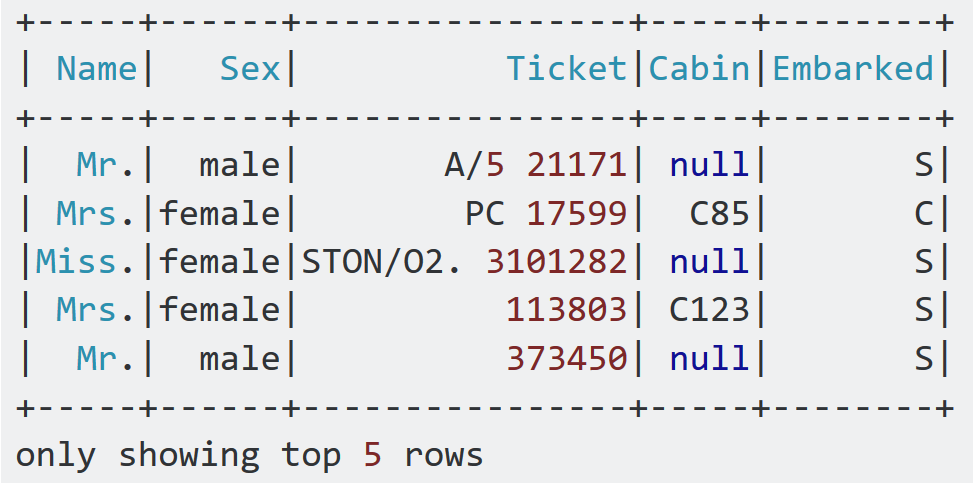Answer to question 5: For many problems, you can start with just one or two hidden layers. This setting will work just fine using two hidden layers with the same total number of neurons (continue reading to get an idea about a number of neurons) in roughly the same amount of training time. Now let's see some naïve estimation about setting the number of hidden layers:

• 0: Only capable of representing linear separable functions
• 1: Can approximate any function that contains a continuous mapping from one finite space to another
• 2: Can represent an arbitrary decision boundary to arbitrary accuracy

However, for a more complex problem, you can gradually ramp up the number of hidden layers, until you start overfitting the training set. Nevertheless, you can try increasing the number of neurons gradually until the network starts overfitting. This means the upper bound on the number of hidden neurons that will not result in overfitting is:In the preceding equation:

• Ni = number of input neurons
• No = number of output neurons
• Ns = number of samples in training dataset
• α = an arbitrary scaling factor, usually 2-10

Note that the preceding equation does not come from any research but from my personal working experience.

Answer to question 6: Of course, we can. We can cross-validate the training and create a grid search technique for finding the best hyperparameters. Let's give it a try.

First, we have the layers defined. Unfortunately, we cannot cross-validate layers. Probably, it's either a bug or made intentionally by the Spark guys. So we stick to a single layering:

`int[] layers = new int[] {10, 16, 16, 2};`

Then we create the trainer and set only the layer and seed parameters:

```MultilayerPerceptronClassifier mlp = new MultilayerPerceptronClassifier()
.setLayers(layers)
.setSeed(1234L);```

We search through the MLP's different hyperparameters for the best model:

```ParamMap[] paramGrid = new ParamGridBuilder()
.build();
MulticlassClassificationEvaluator evaluator = new MulticlassClassificationEvaluator()
.setLabelCol("label")
.setPredictionCol("prediction");```

We then set up the cross-validator and perform 10-fold cross-validation:

```int numFolds = 10;
CrossValidator crossval = new CrossValidator()
.setEstimator(mlp)
.setEvaluator(evaluator)
.setEstimatorParamMaps(paramGrid)
.setNumFolds(numFolds);```

Then we perform training using the cross-validated model:

`CrossValidatorModel cvModel = crossval.fit(trainingData);`

Finally, we evaluate the cross-validated model on the test set, as follows:

`Dataset<Row> predictions = cvModel.transform(validationData);`

Now we can compute and show the performance metrics, similar to our previous example:

```double accuracy = evaluator1.evaluate(predictions);
double precision = evaluator2.evaluate(predictions);
double recall = evaluator3.evaluate(predictions);
double f1 = evaluator4.evaluate(predictions);

// Print the performance metrics
System.out.println("Accuracy = " + accuracy);
System.out.println("Precision = " + precision);
System.out.println("Recall = " + recall);
System.out.println("F1 = " + f1);
System.out.println("Test Error = " + (1 - accuracy));
```
```>>>
A```ccuracy = 0.7810132575757576
Precision = 0.7810132575757576
Recall = 0.7810132575757576
F1 = 0.7810132575757576
Test Error = 0.21898674242424243``````